# Selina Solutions Concise Mathematics Class 6 Chapter 15: Decimal Fractions

Selina Solutions Concise Mathematics Class 6 Chapter 15 Decimal Fractions, help students to grasp the basic concepts, mentioned in this Chapter, comprehensively. Students, who want to strengthen their skills in Mathematics, can use Selina Solutions, prepared by expert teachers in an interactive manner. This also helps them to speed up problem solving abilities in examination and hence, score good marks. Students can get their doubts cleared, by practising Selina Solutions Concise Mathematics Class 6 Chapter 15 Decimal Fractions PDF, from the links which are given below.

Chapter 15 discusses the concepts such as conversion of decimal fractions into fraction and vice versa, decimal places and various mathematical operations on them. Students are advised to practice Selina Solutions regularly to boost their exam preparations.

## Selina Solutions Concise Mathematics Class 6 Chapter 15: Decimal Fractions Download PDF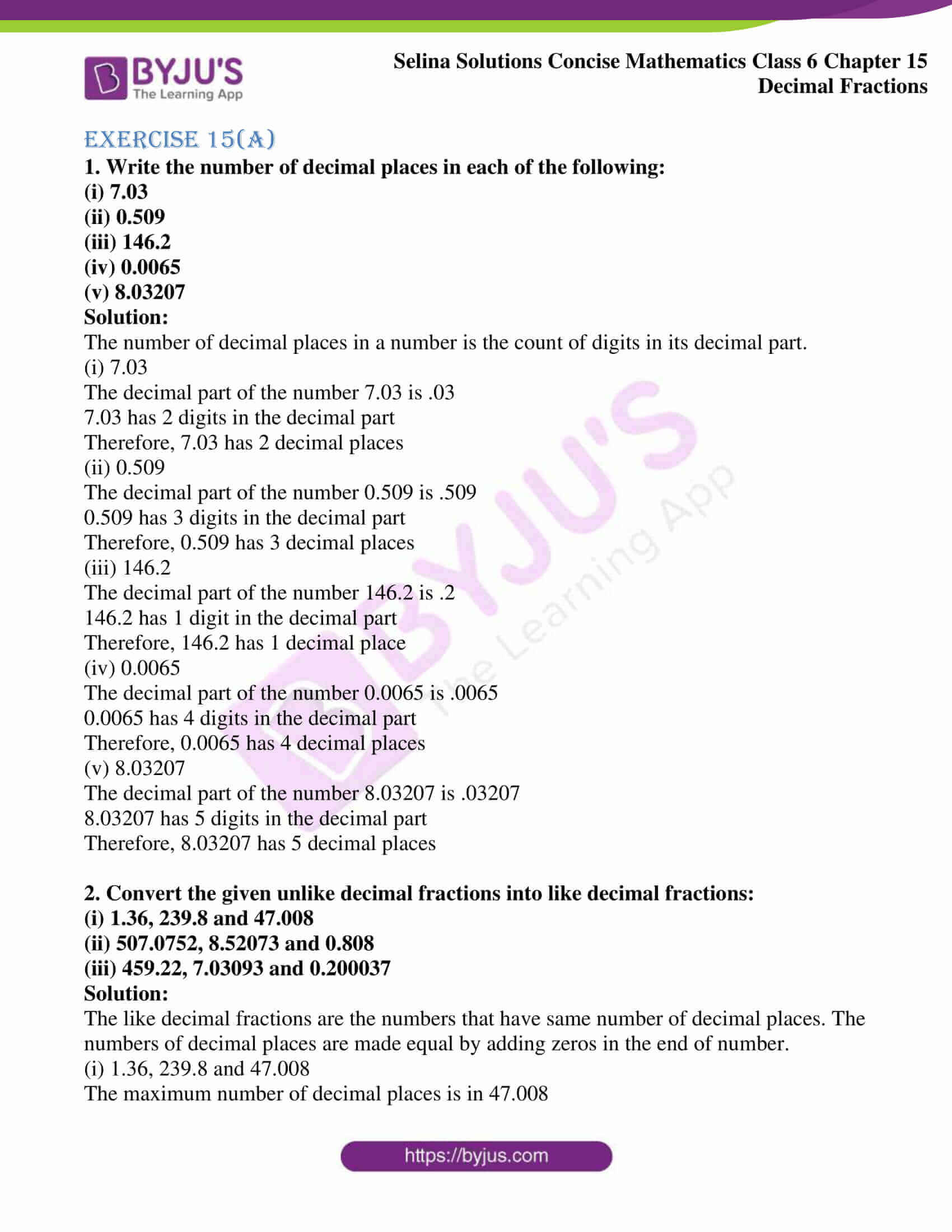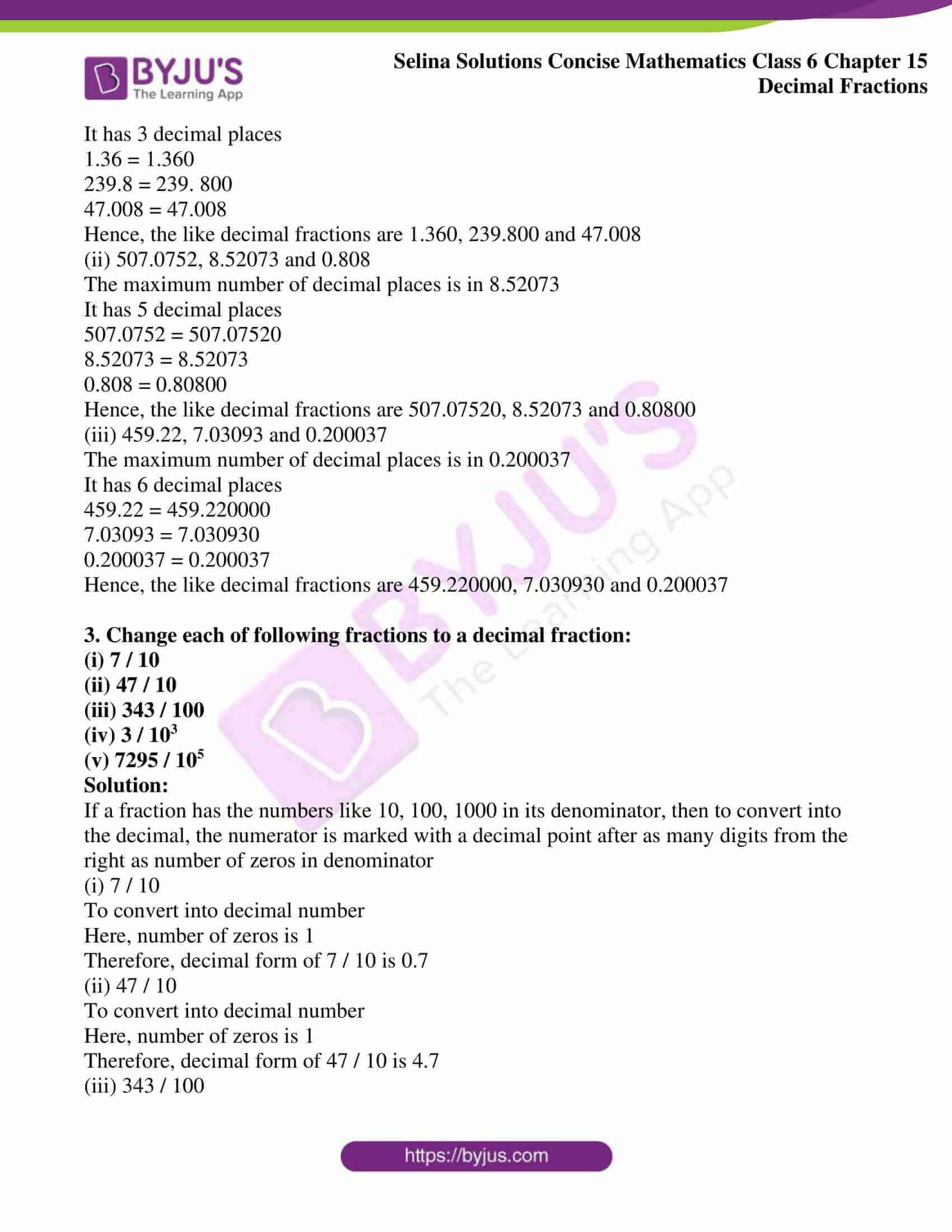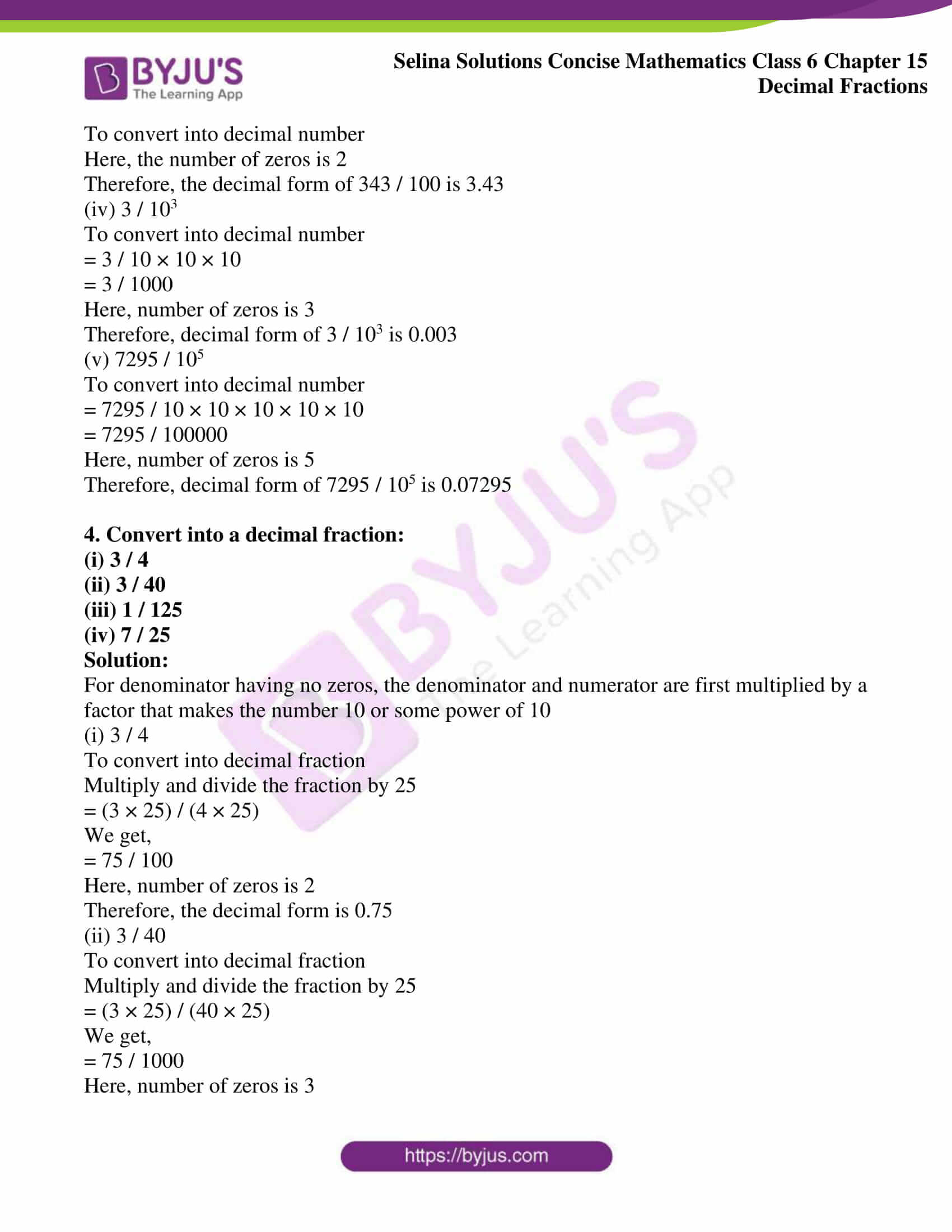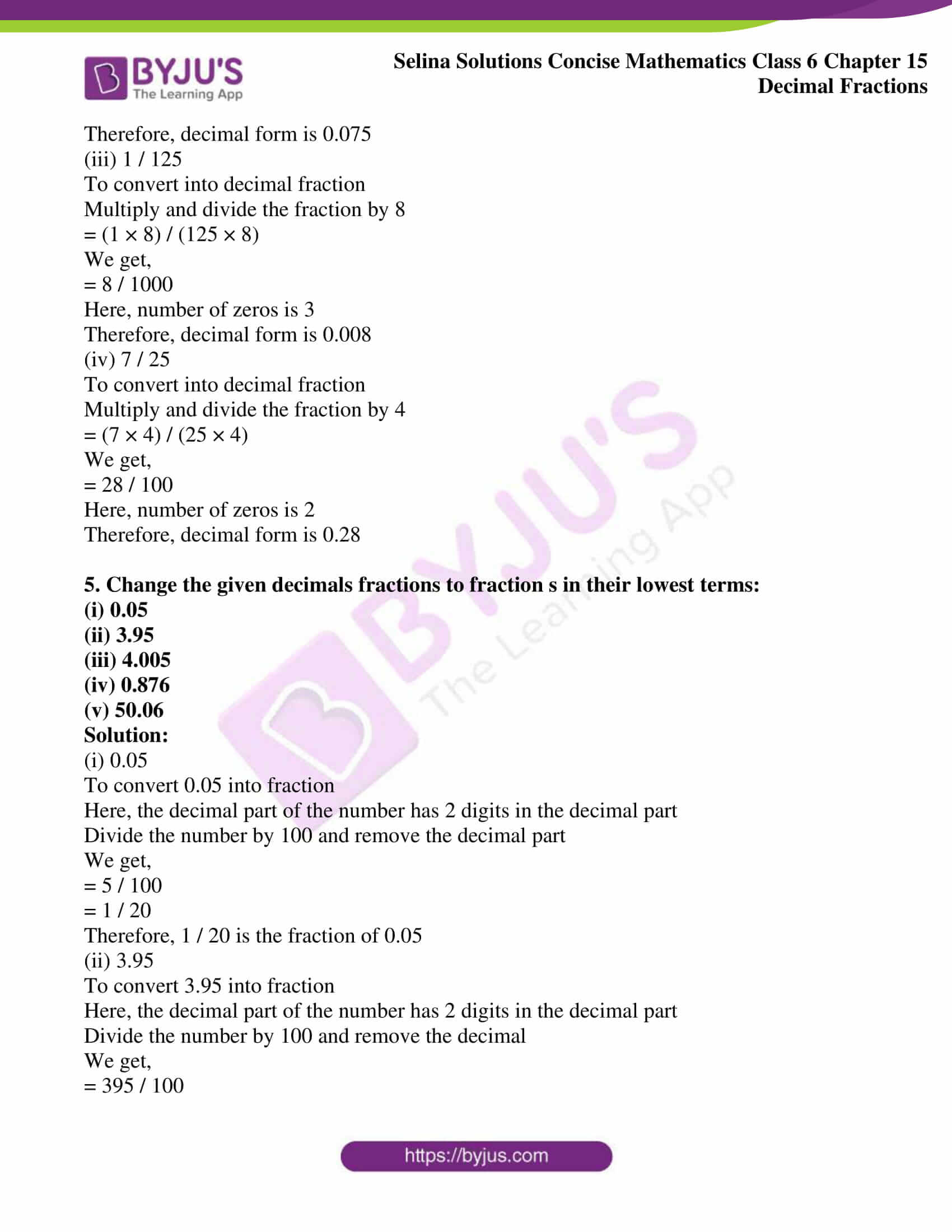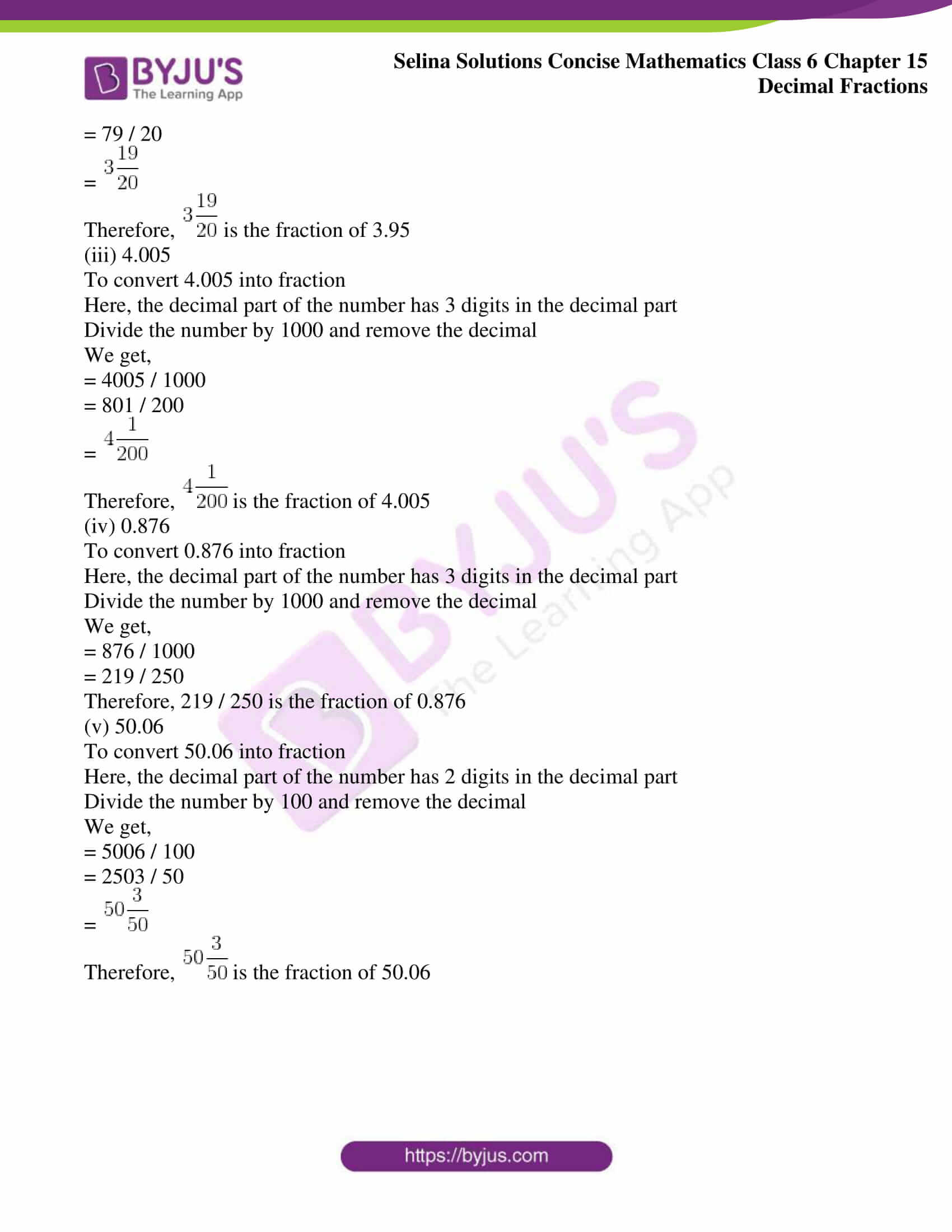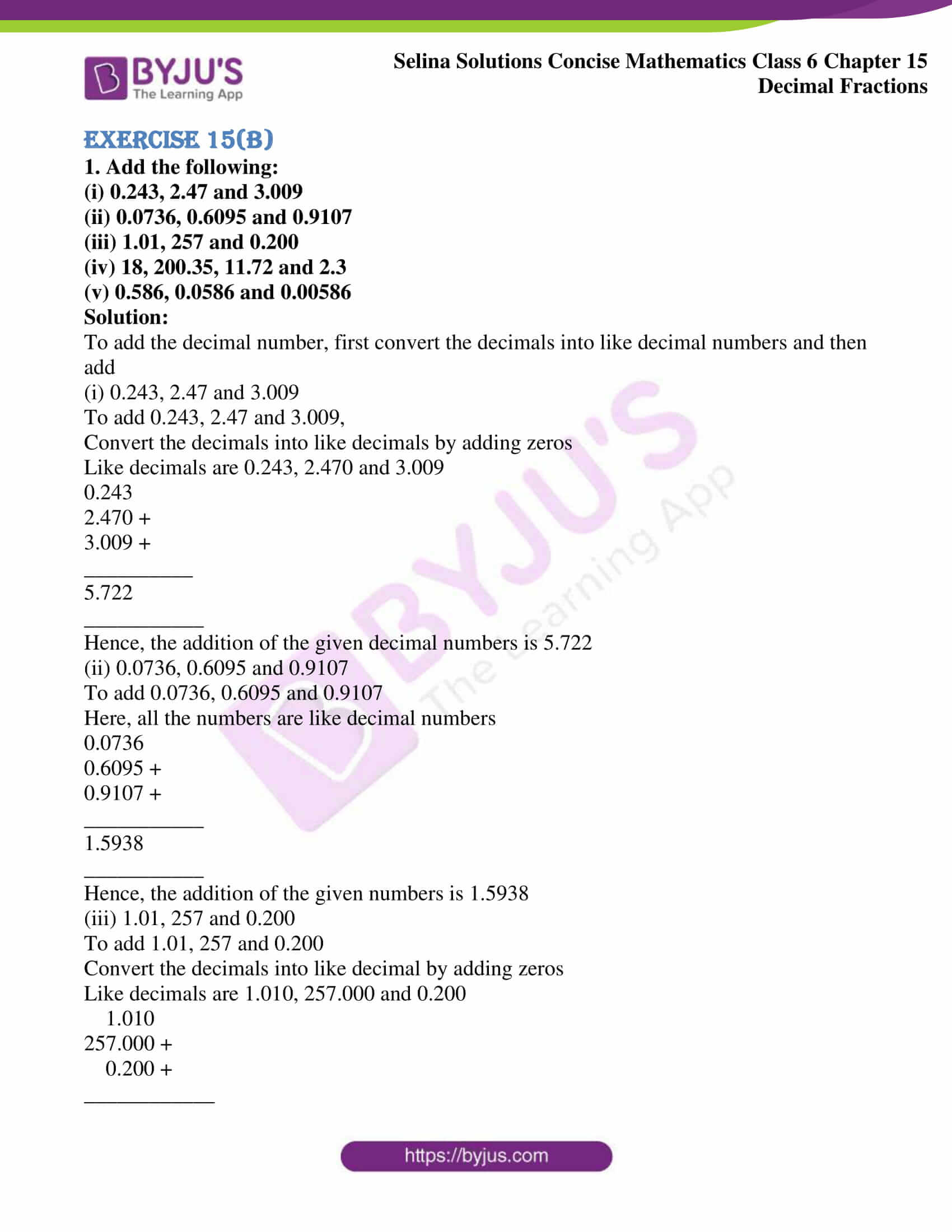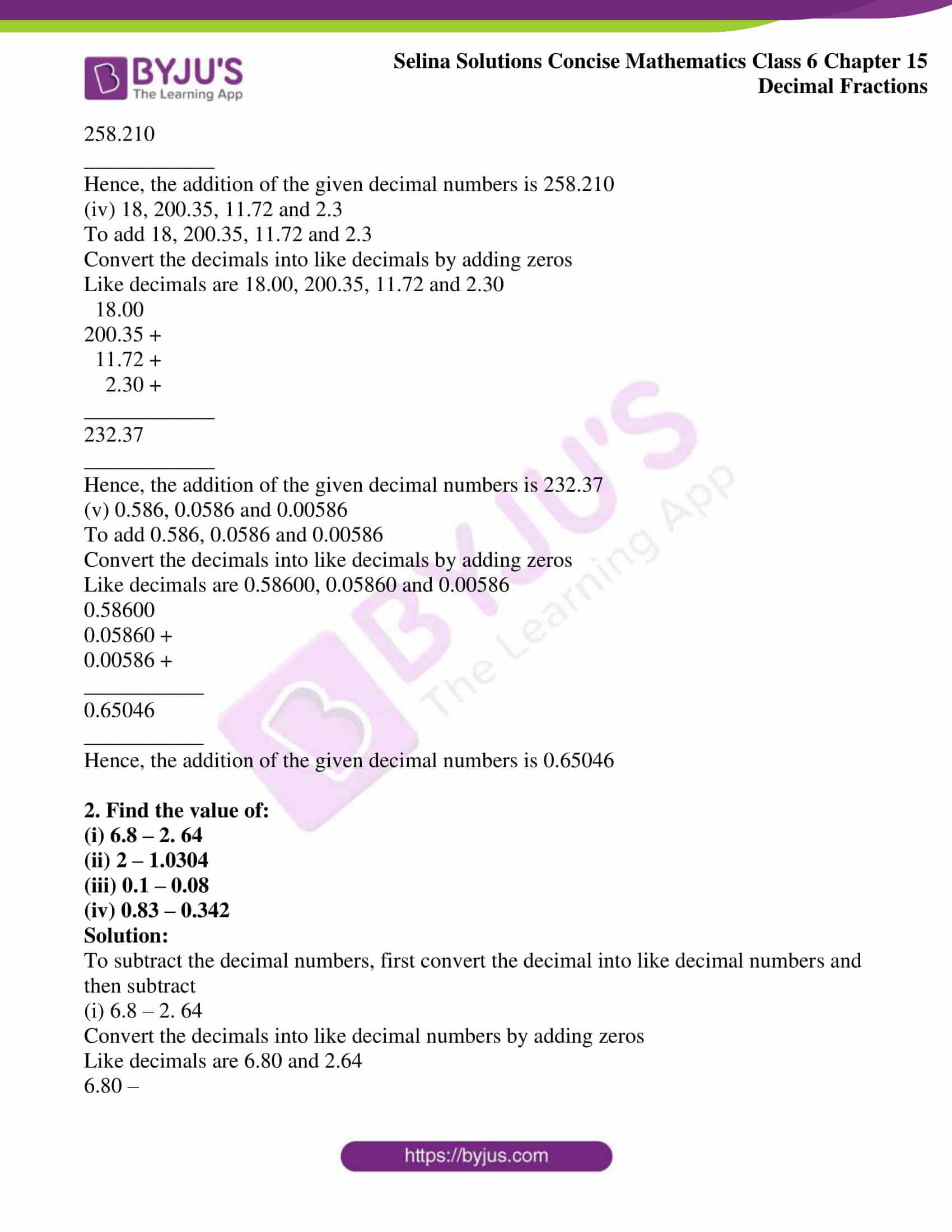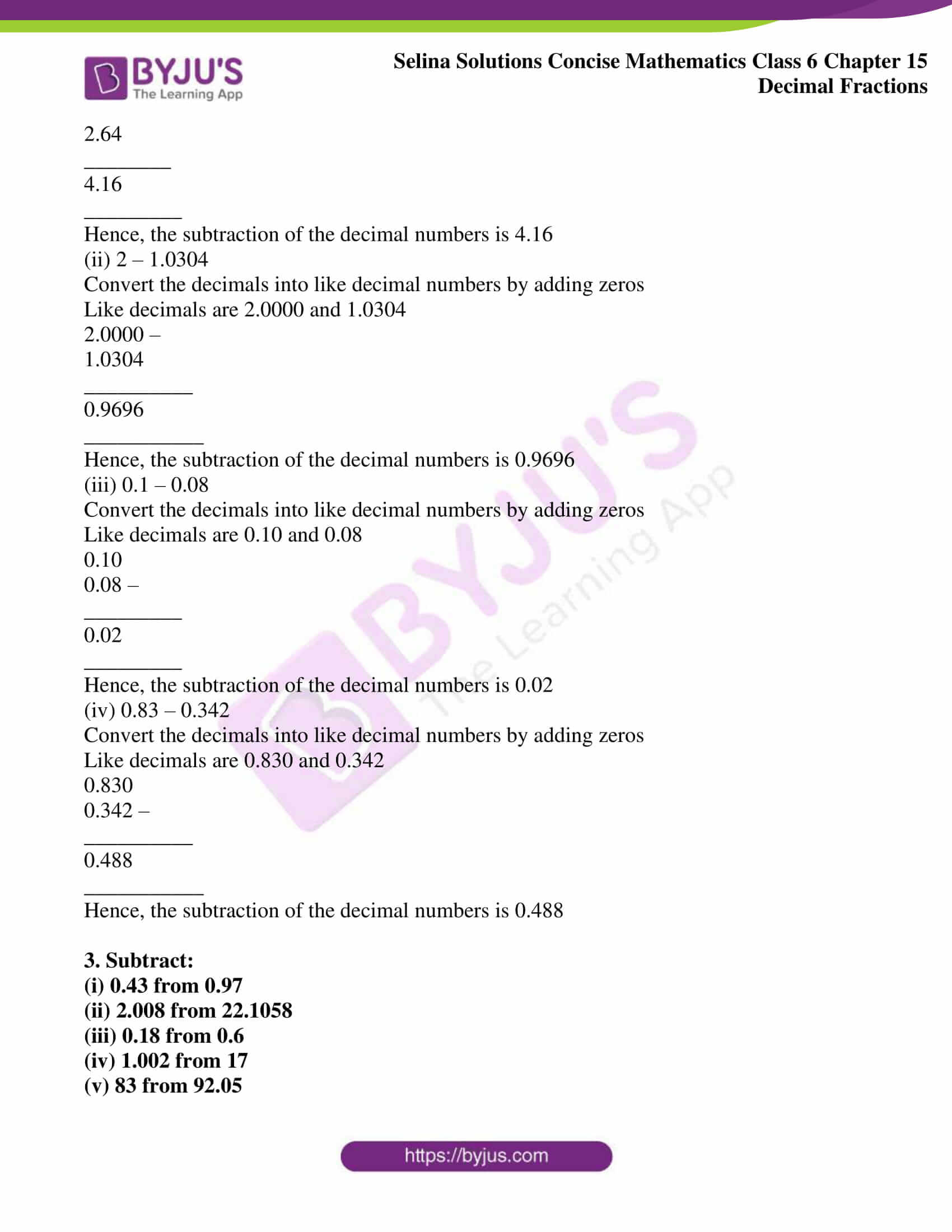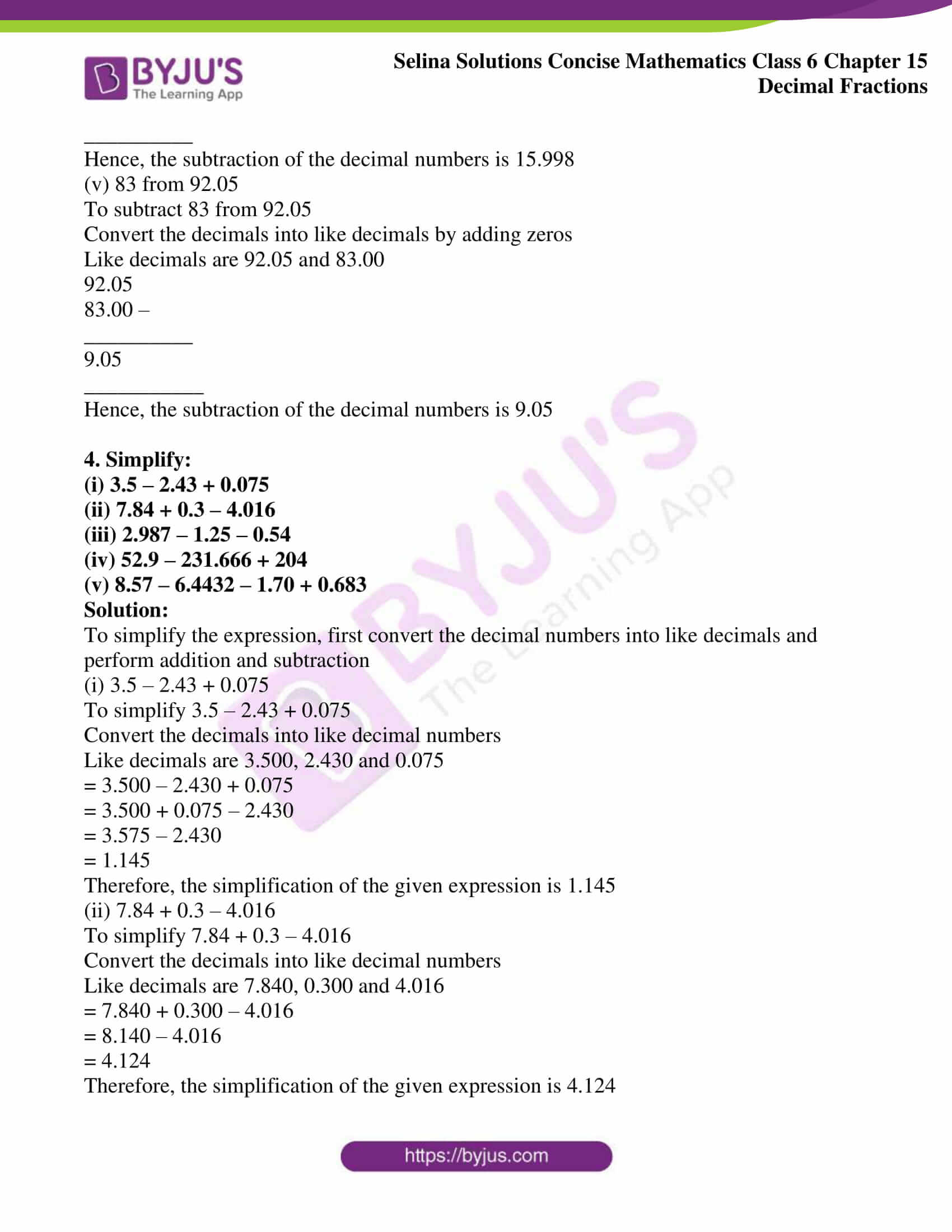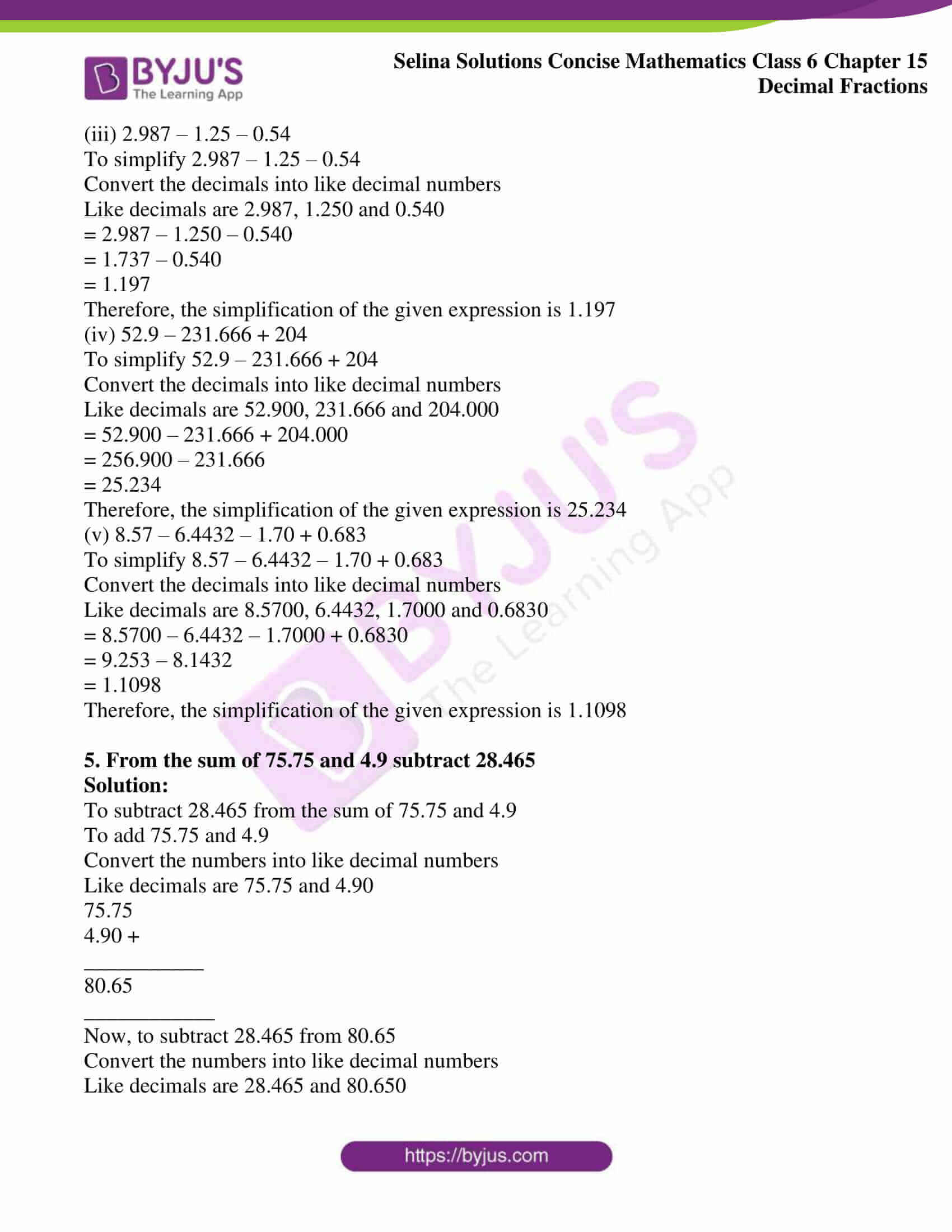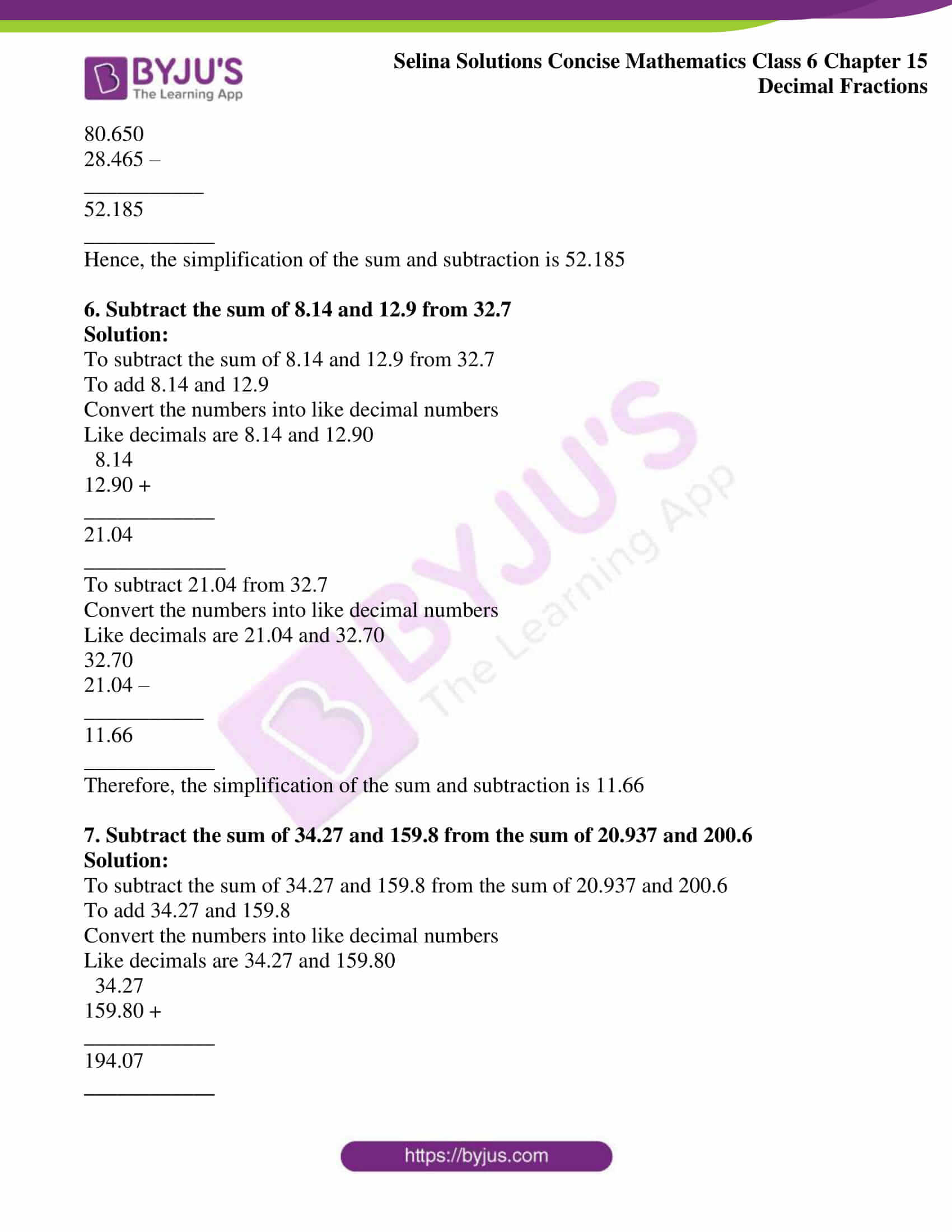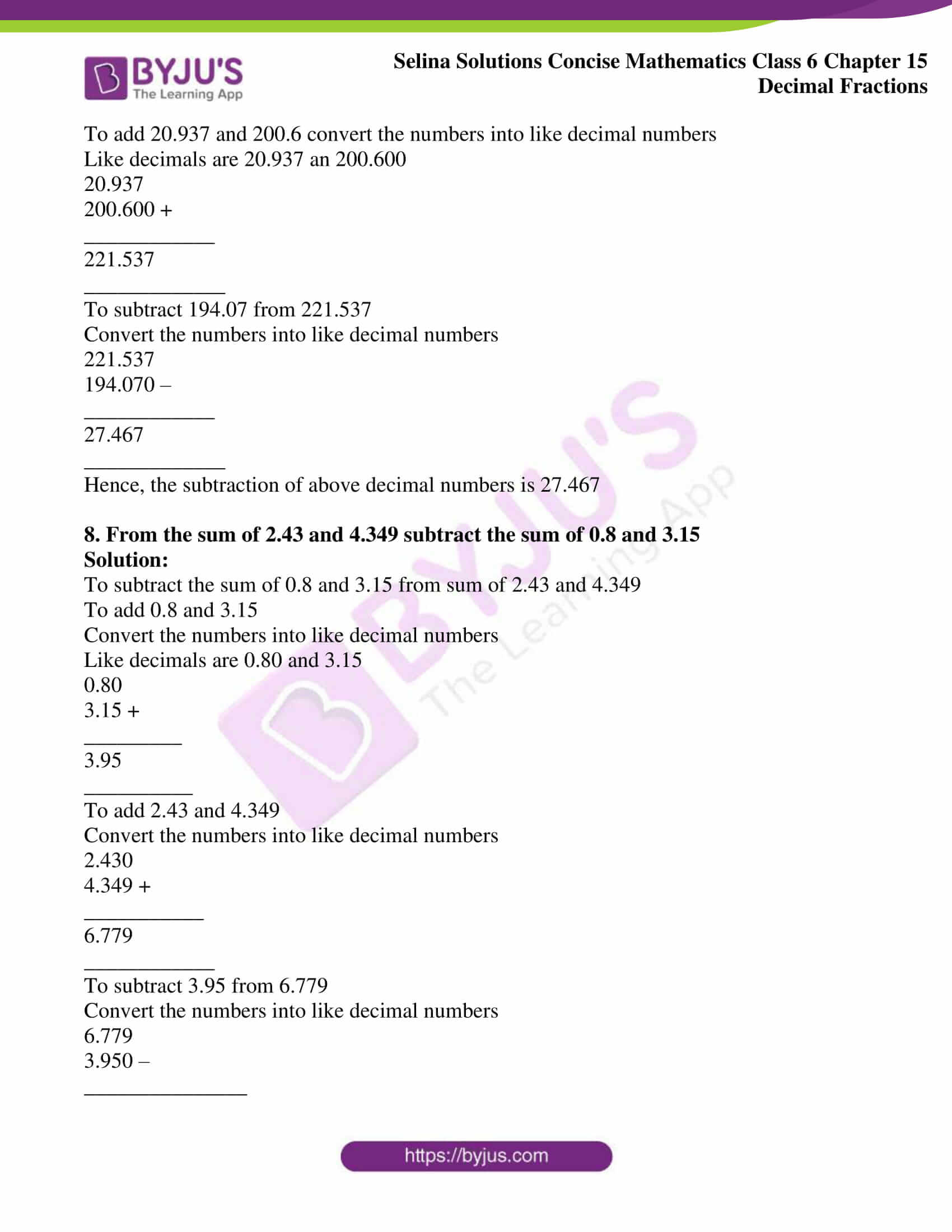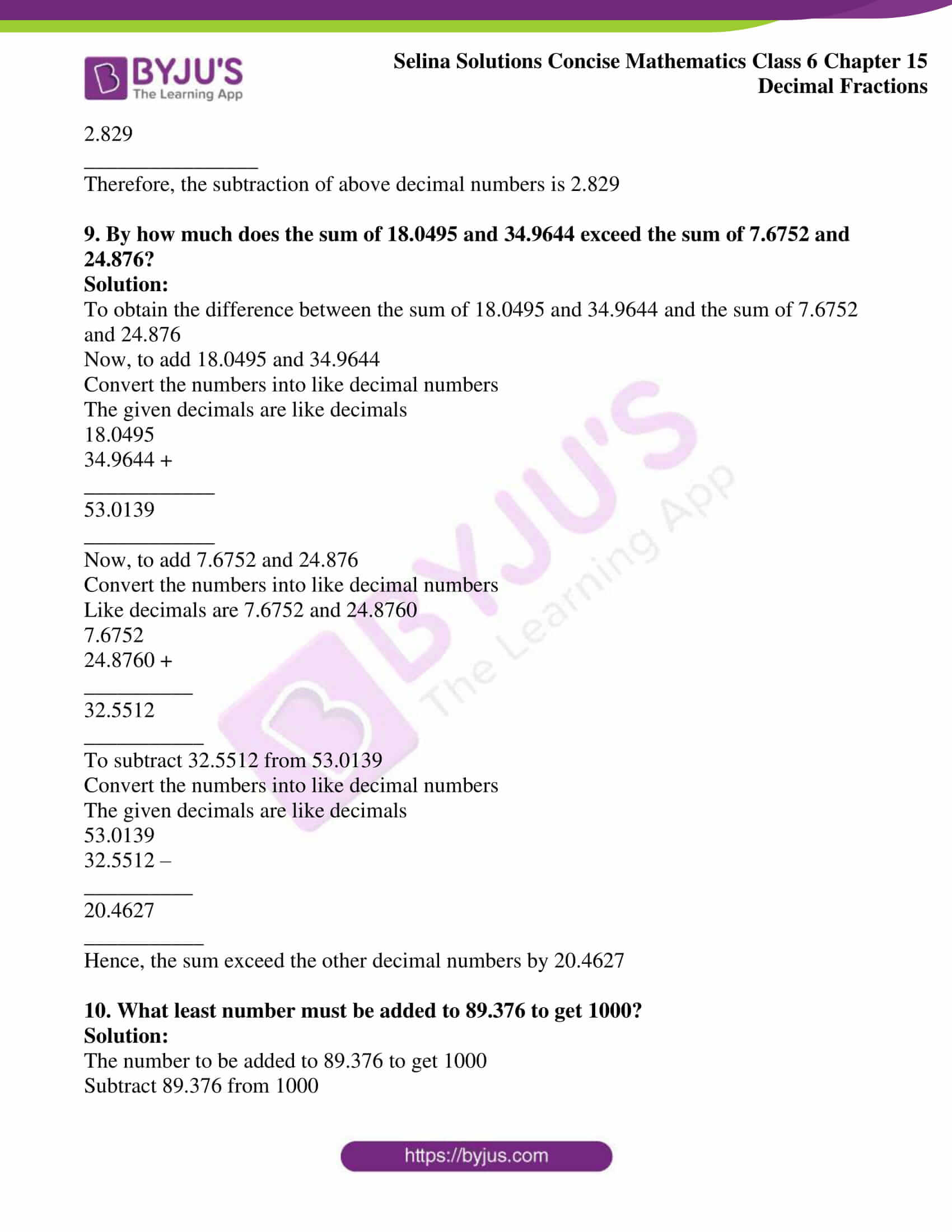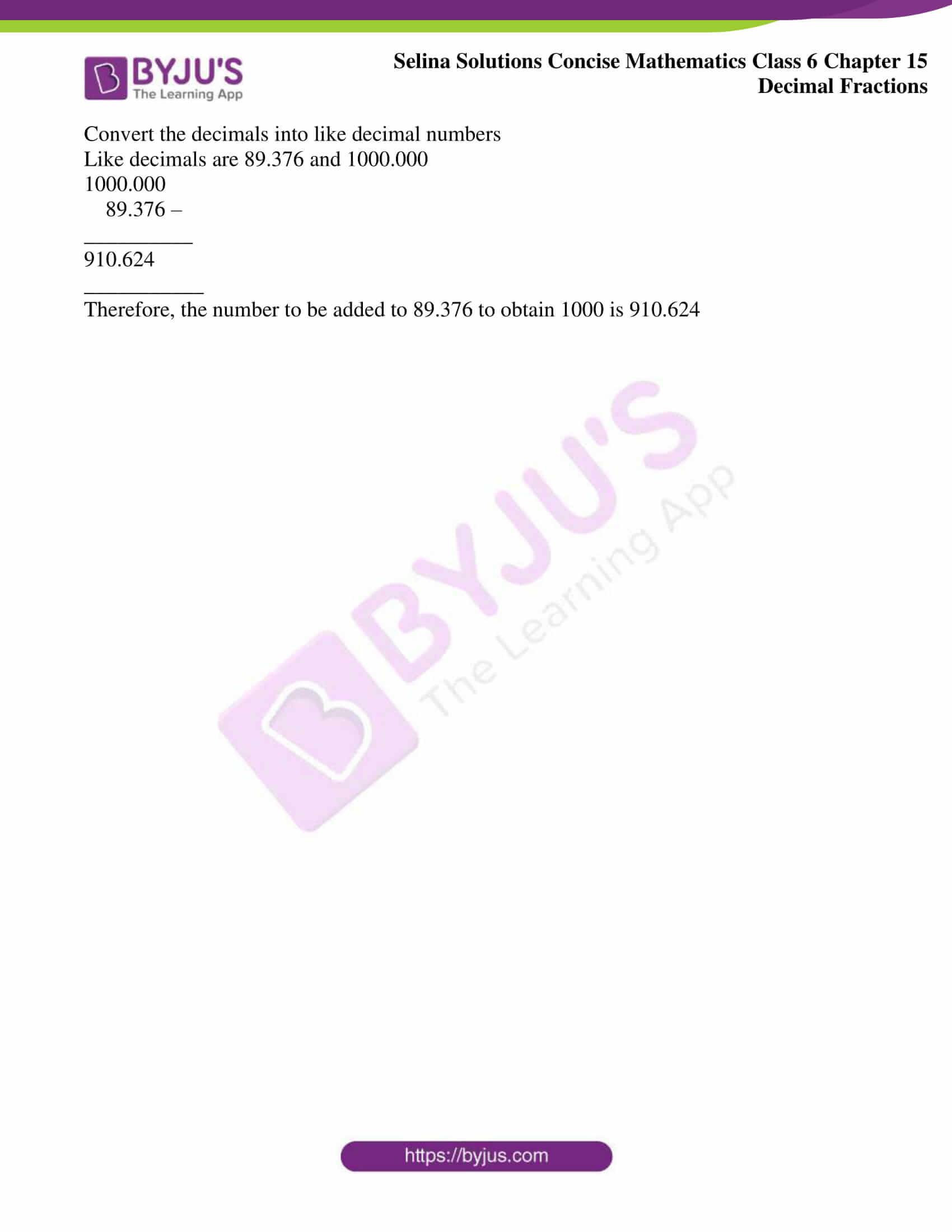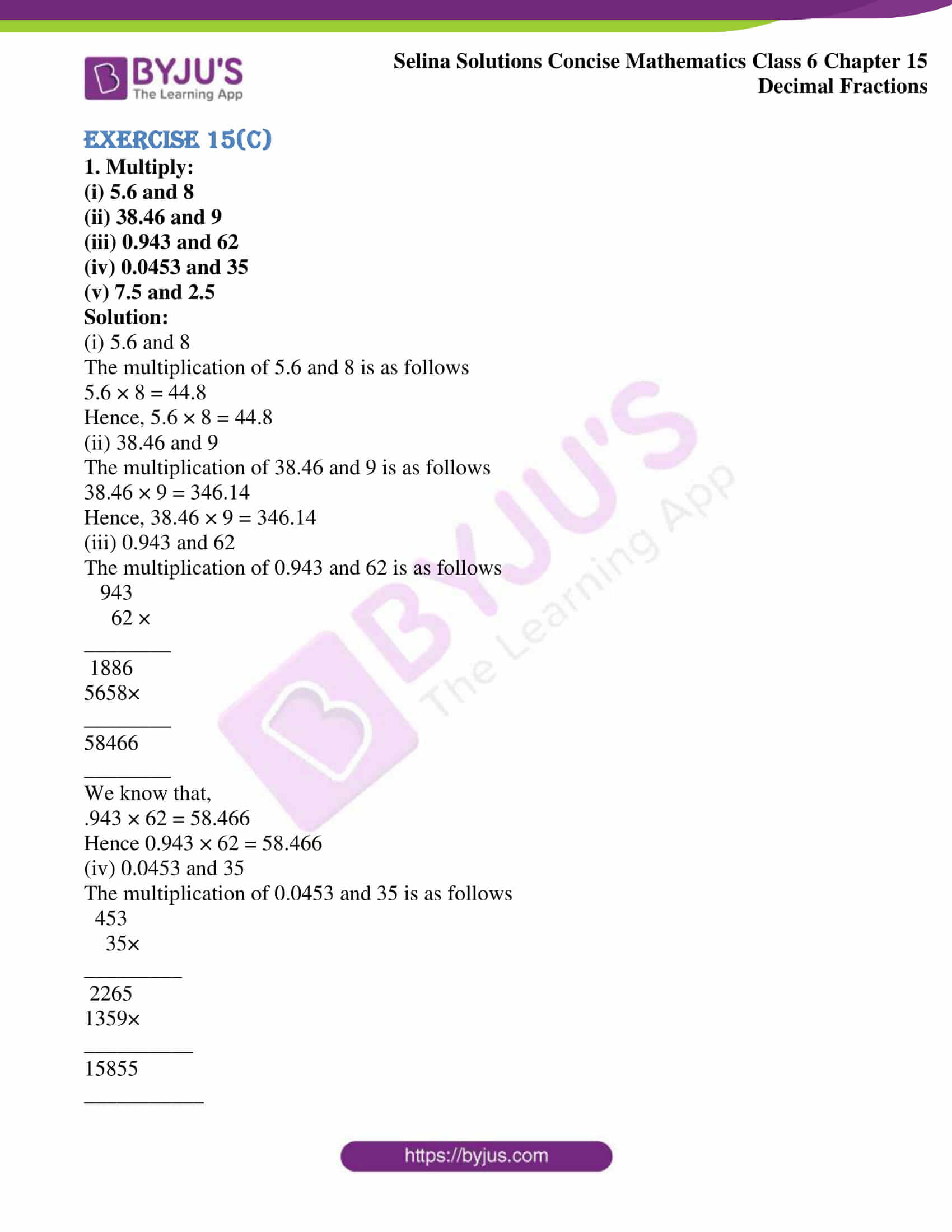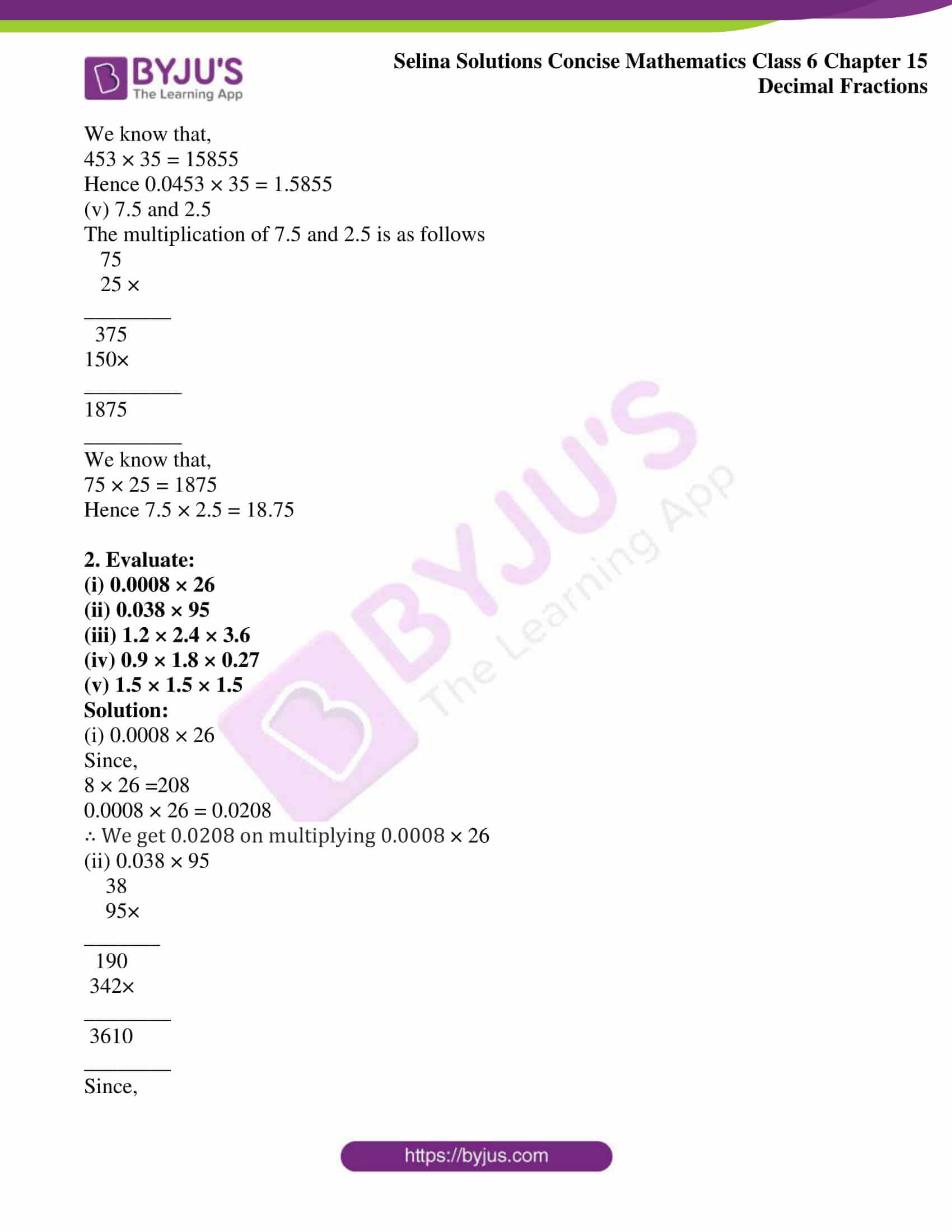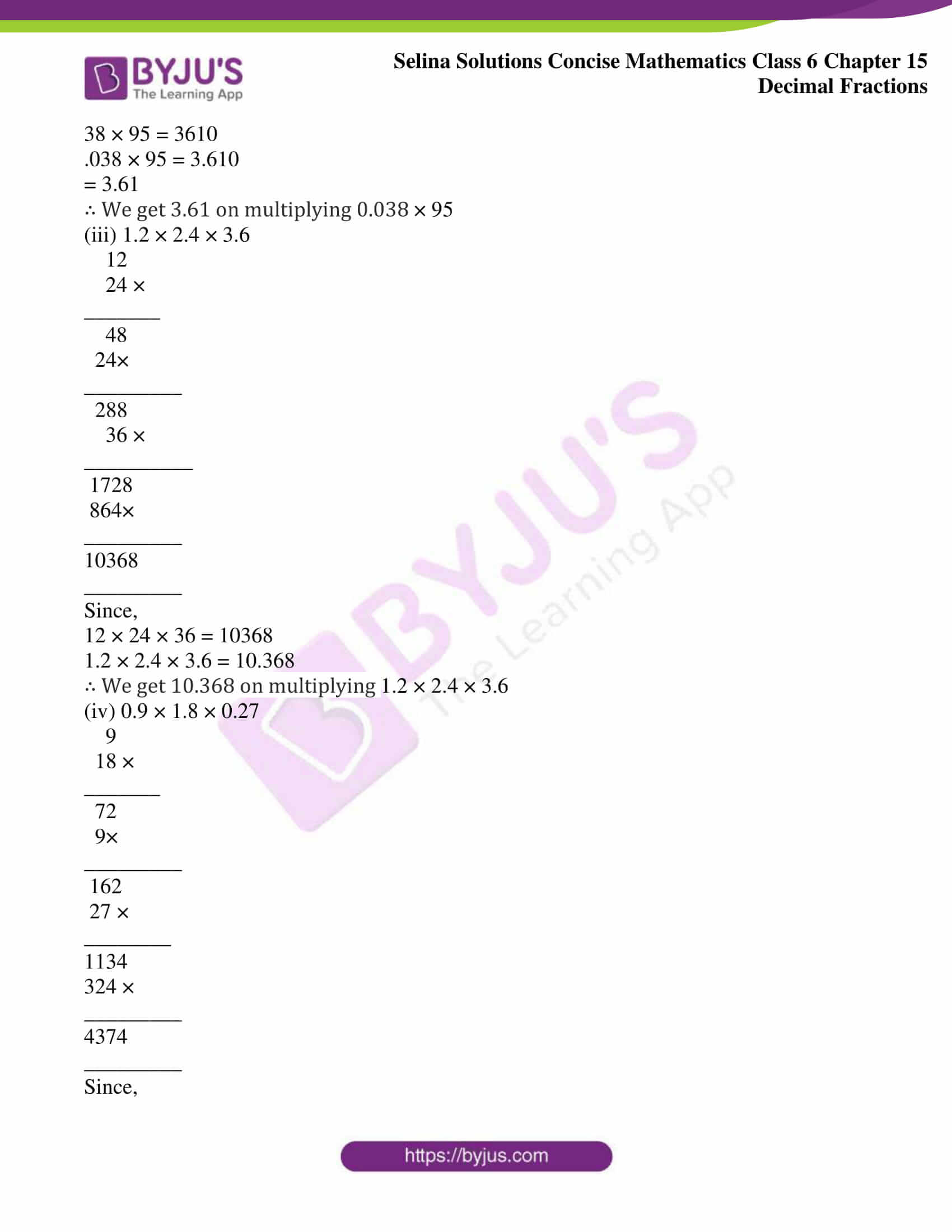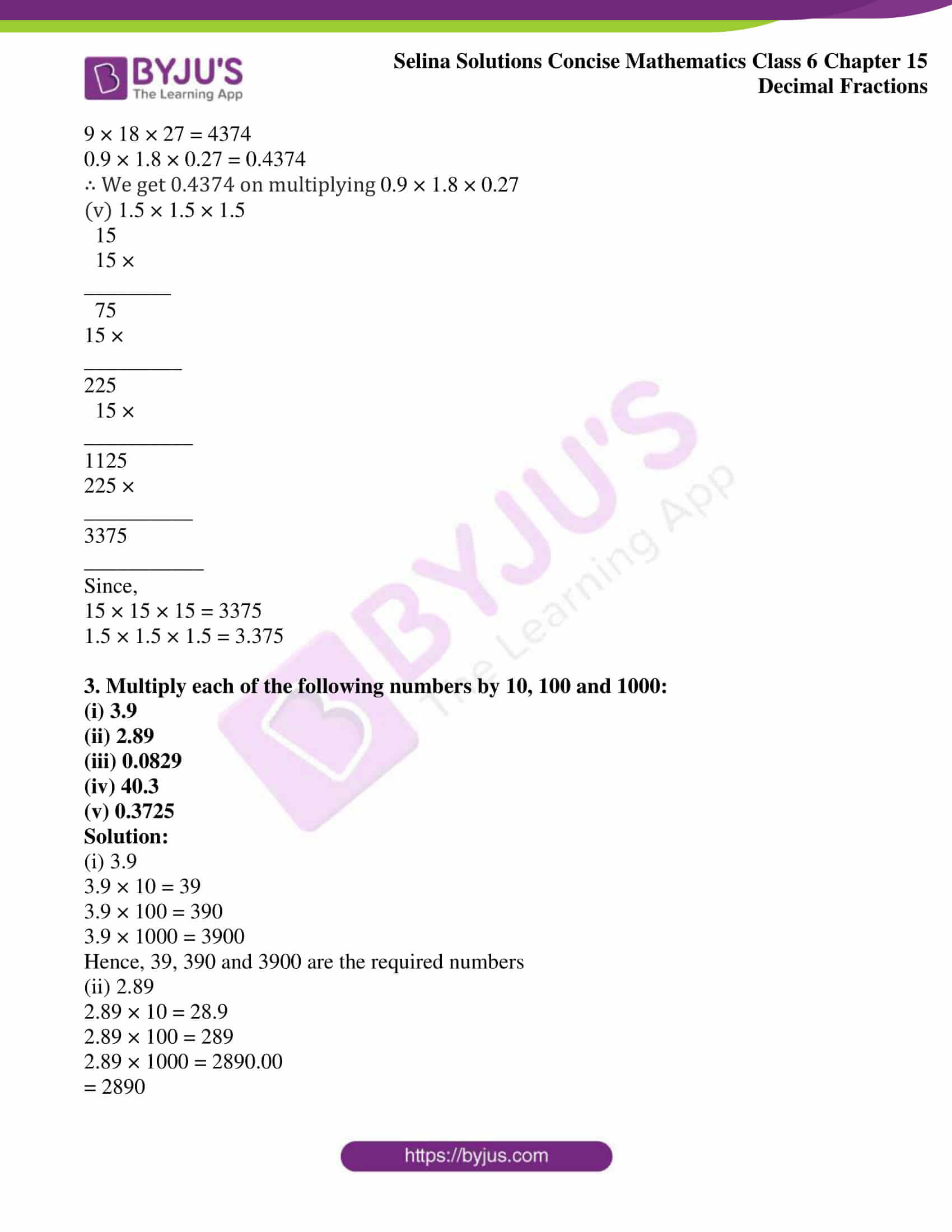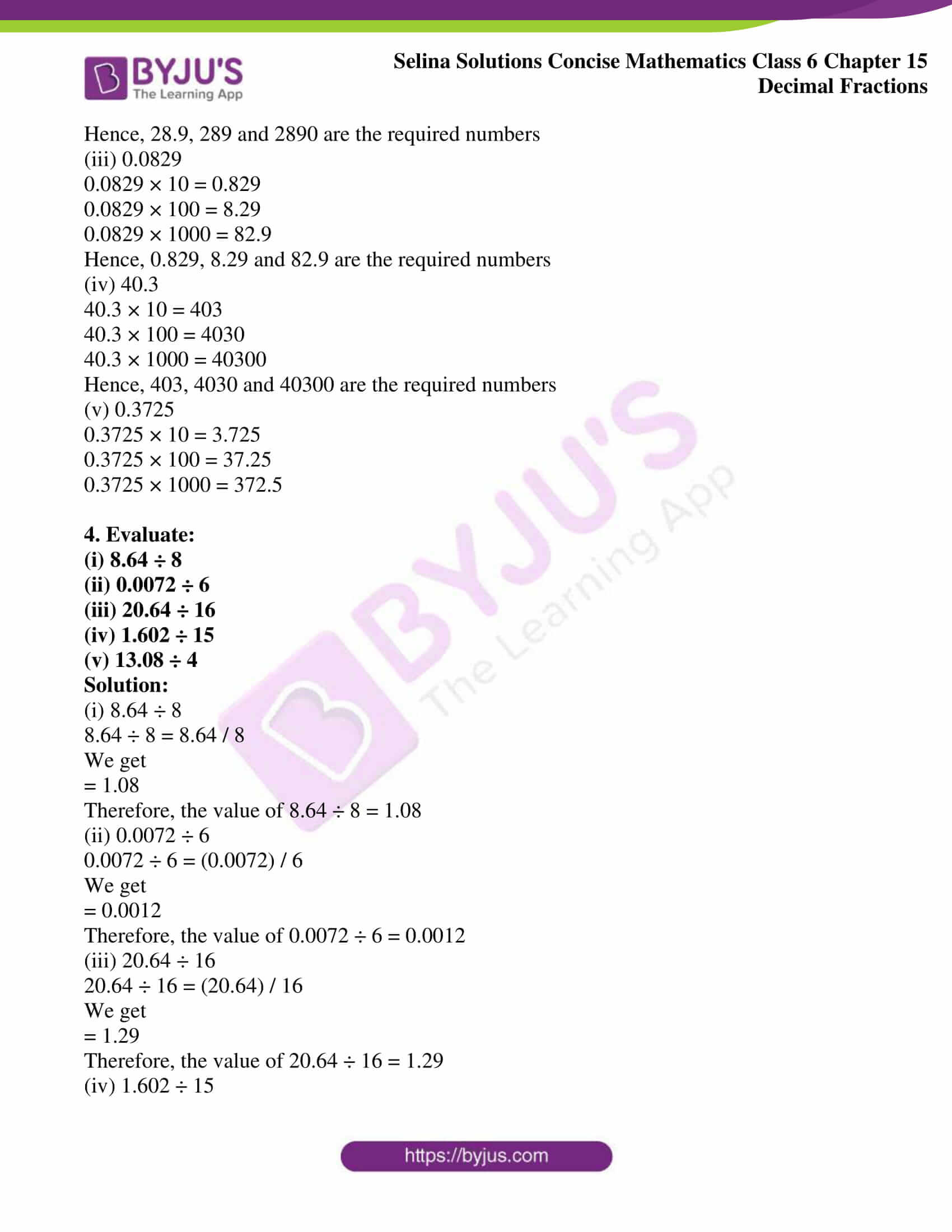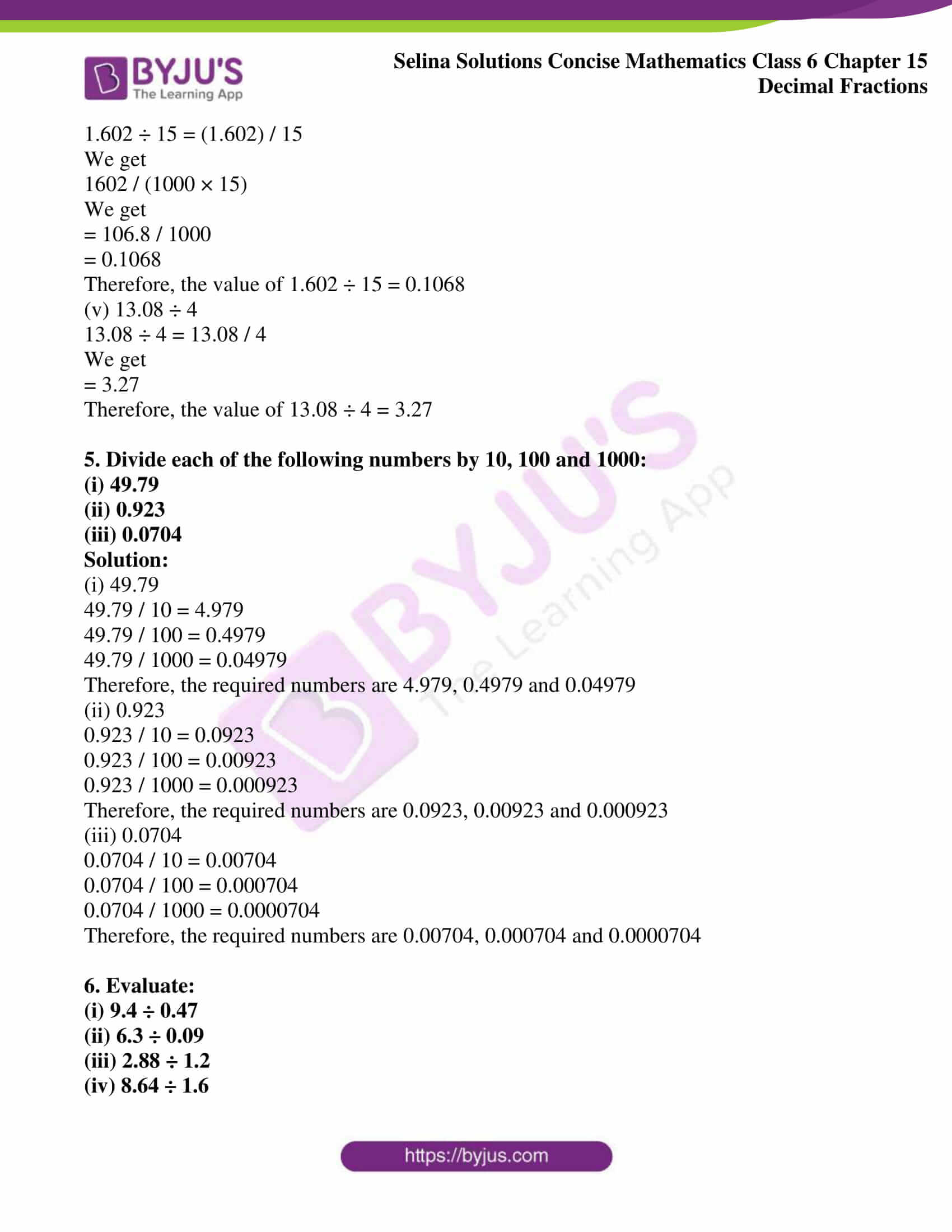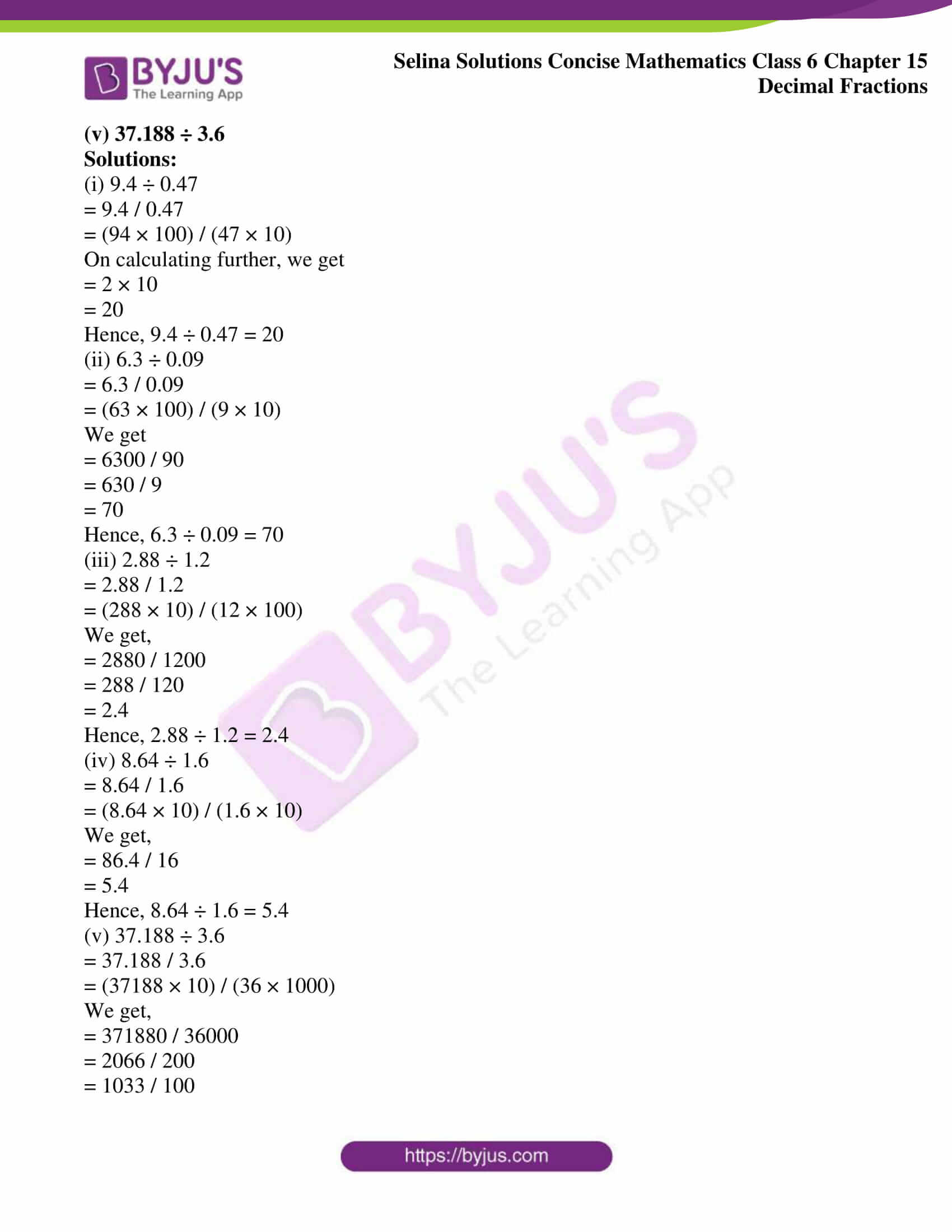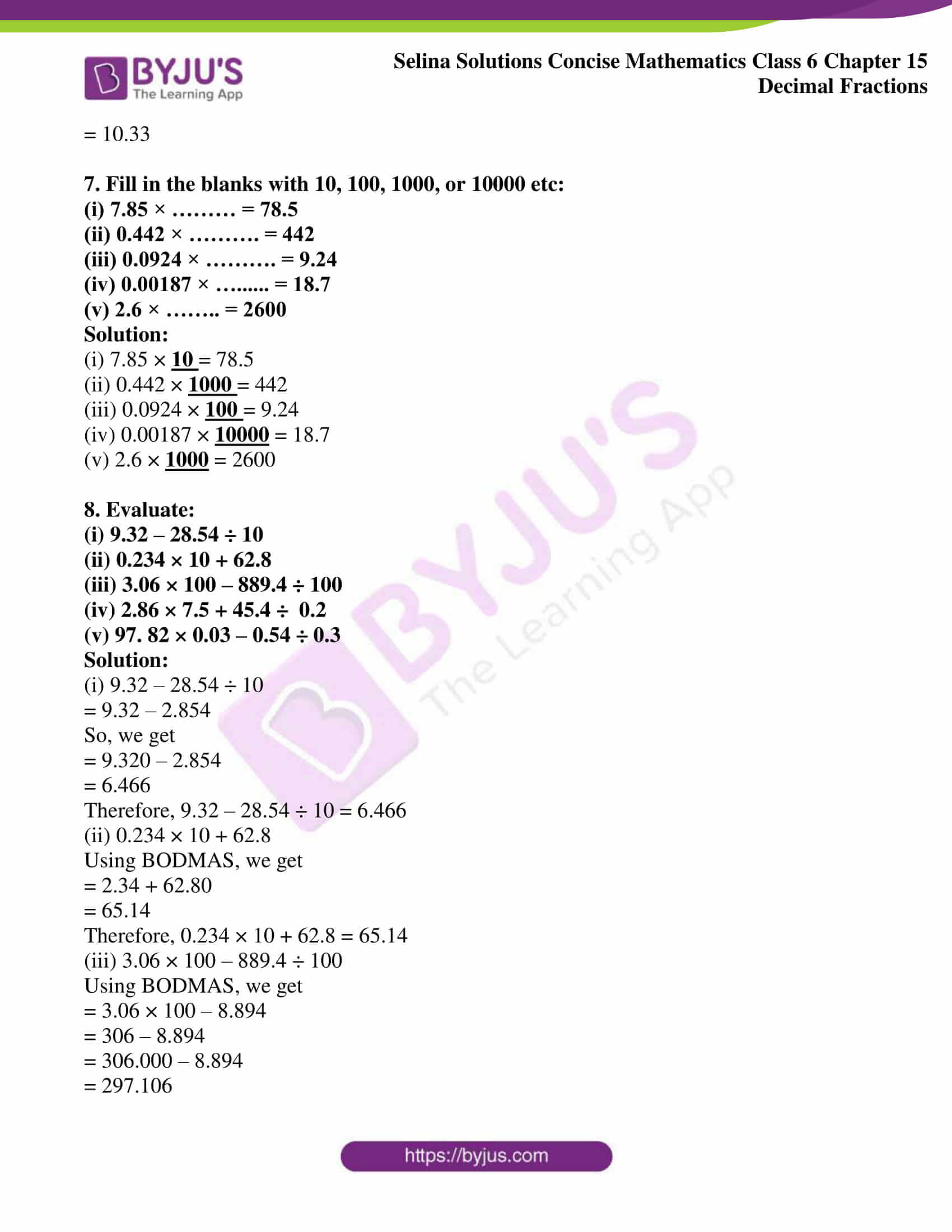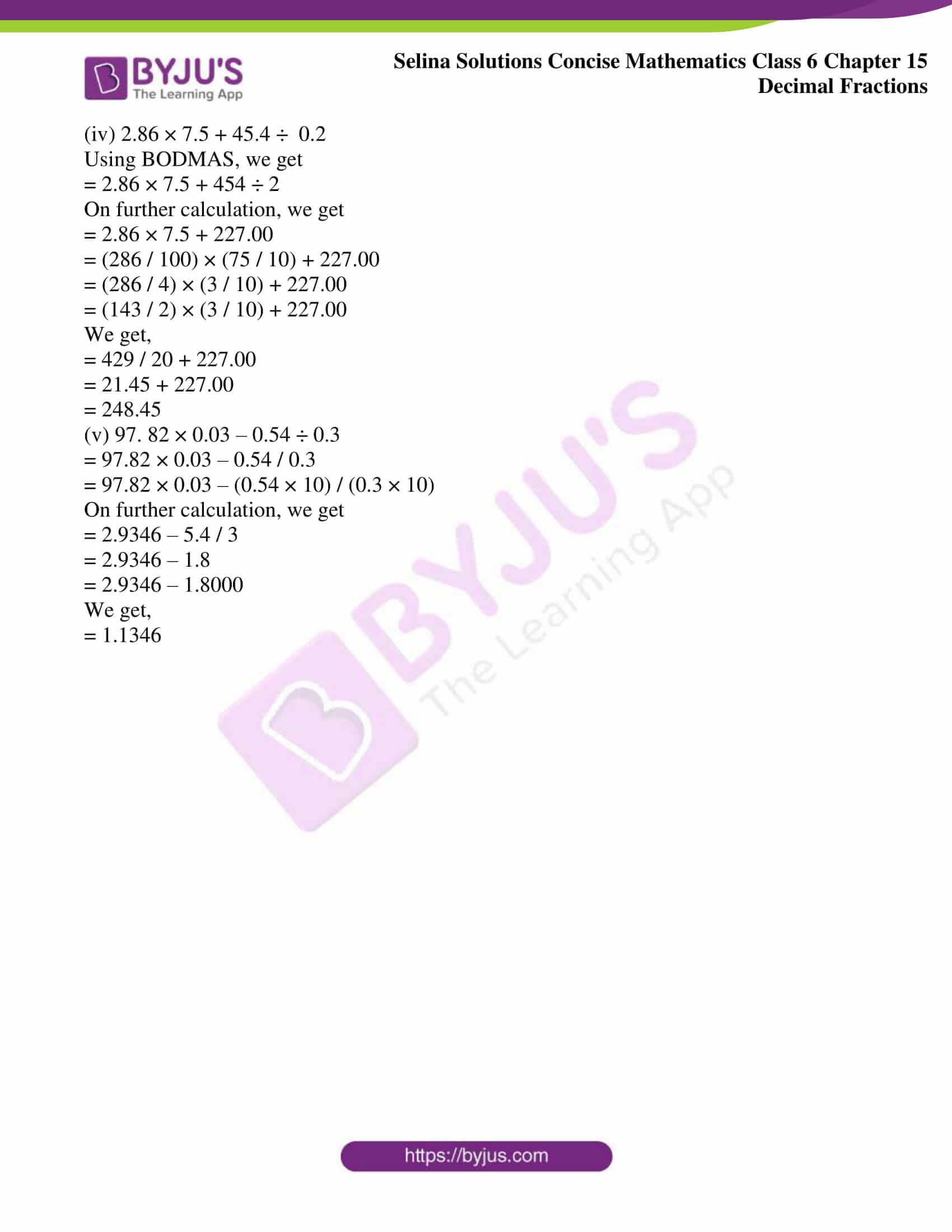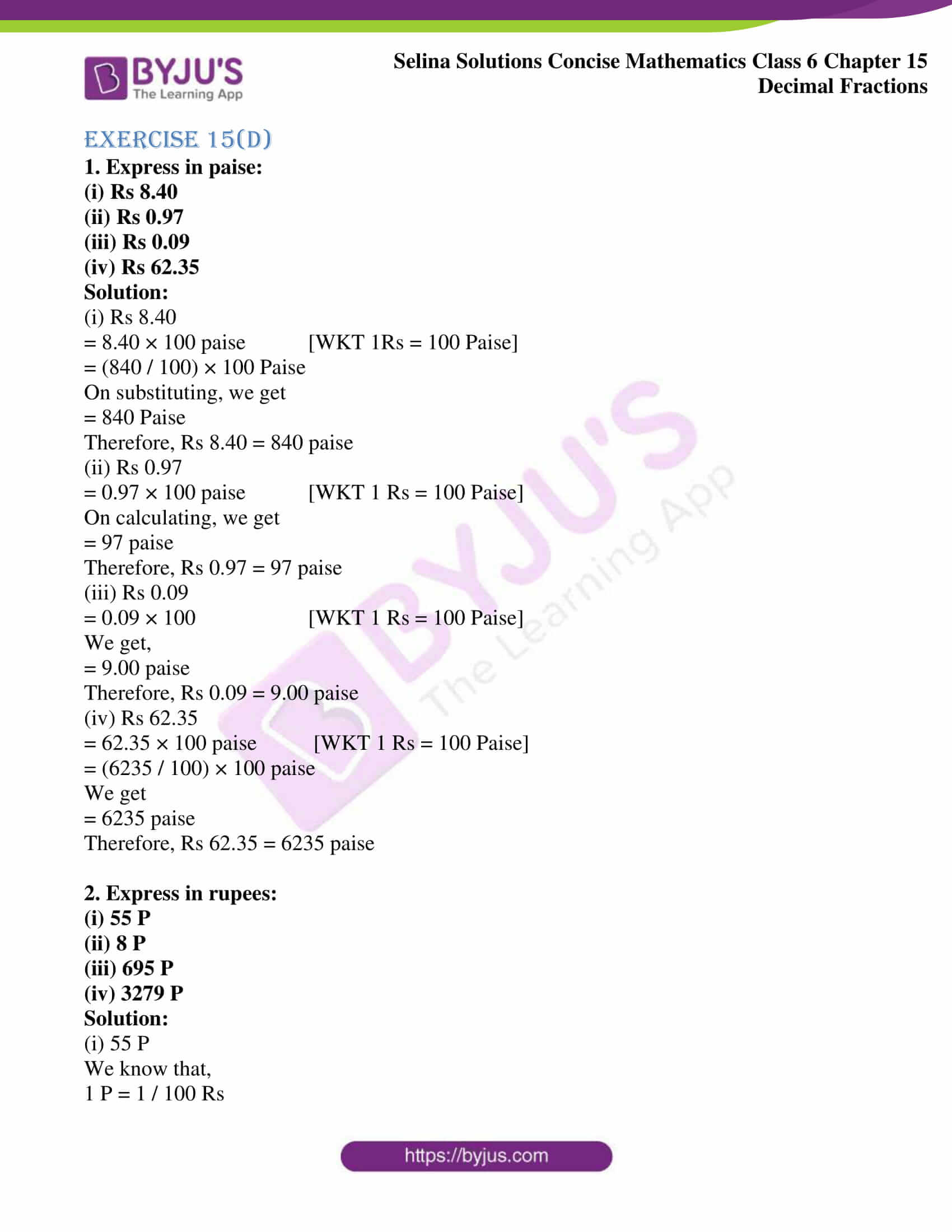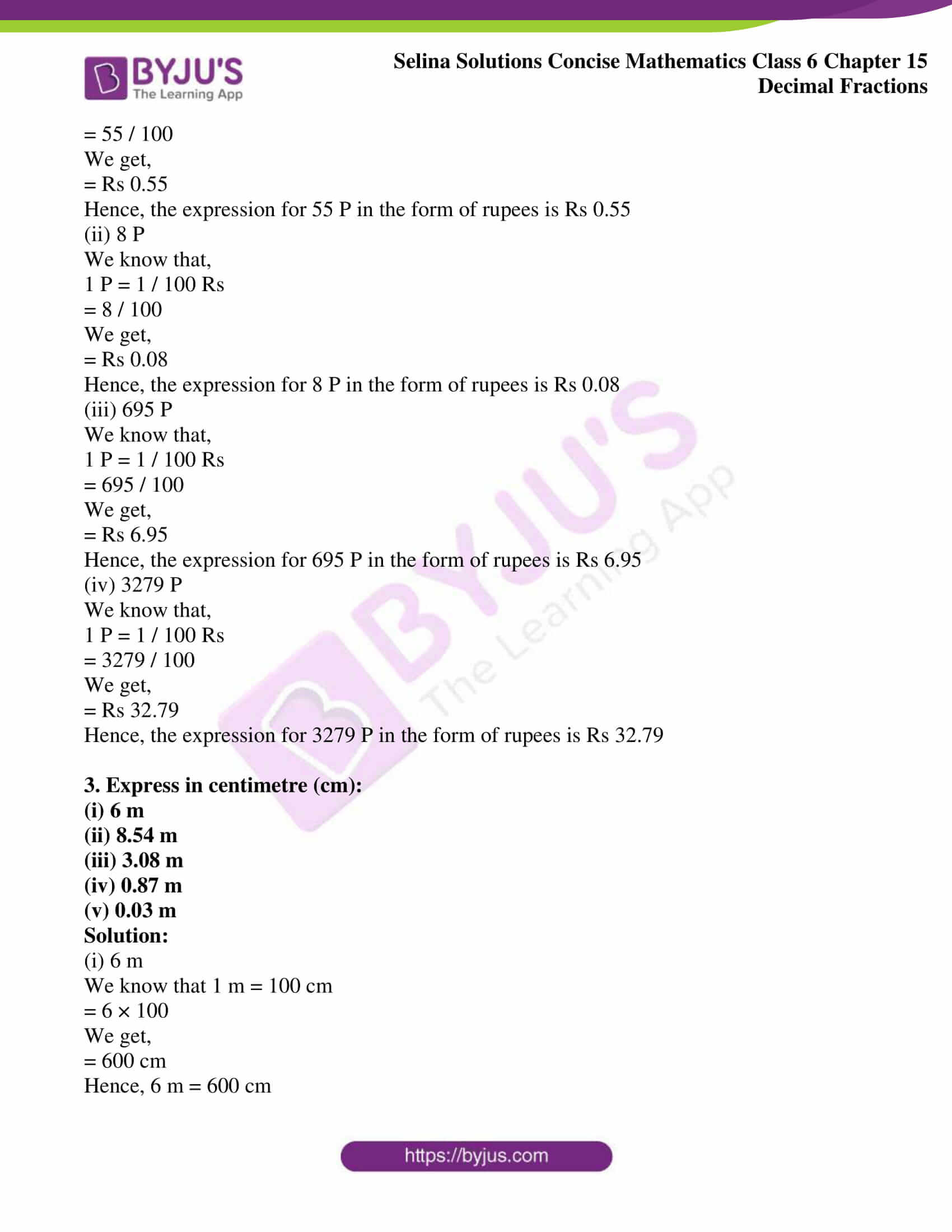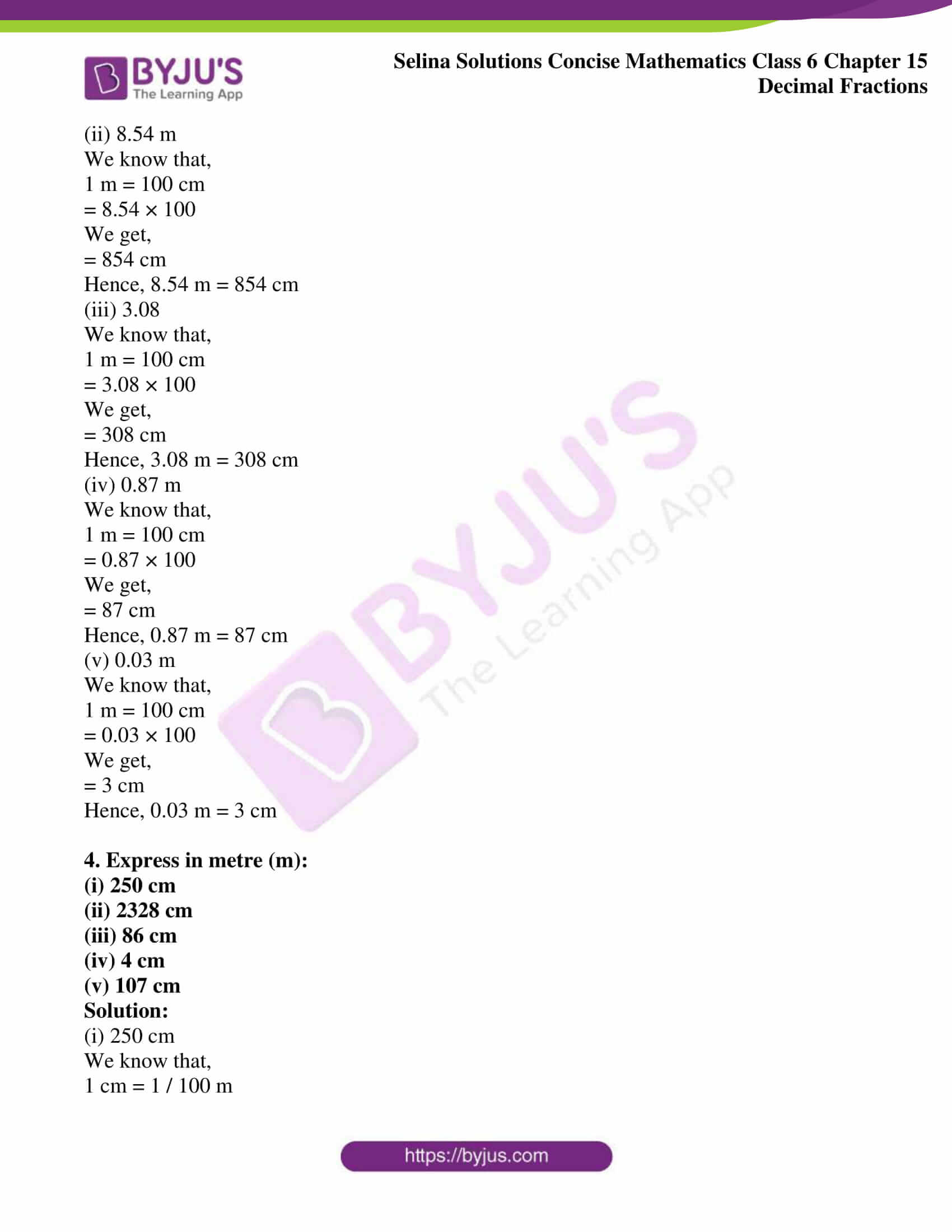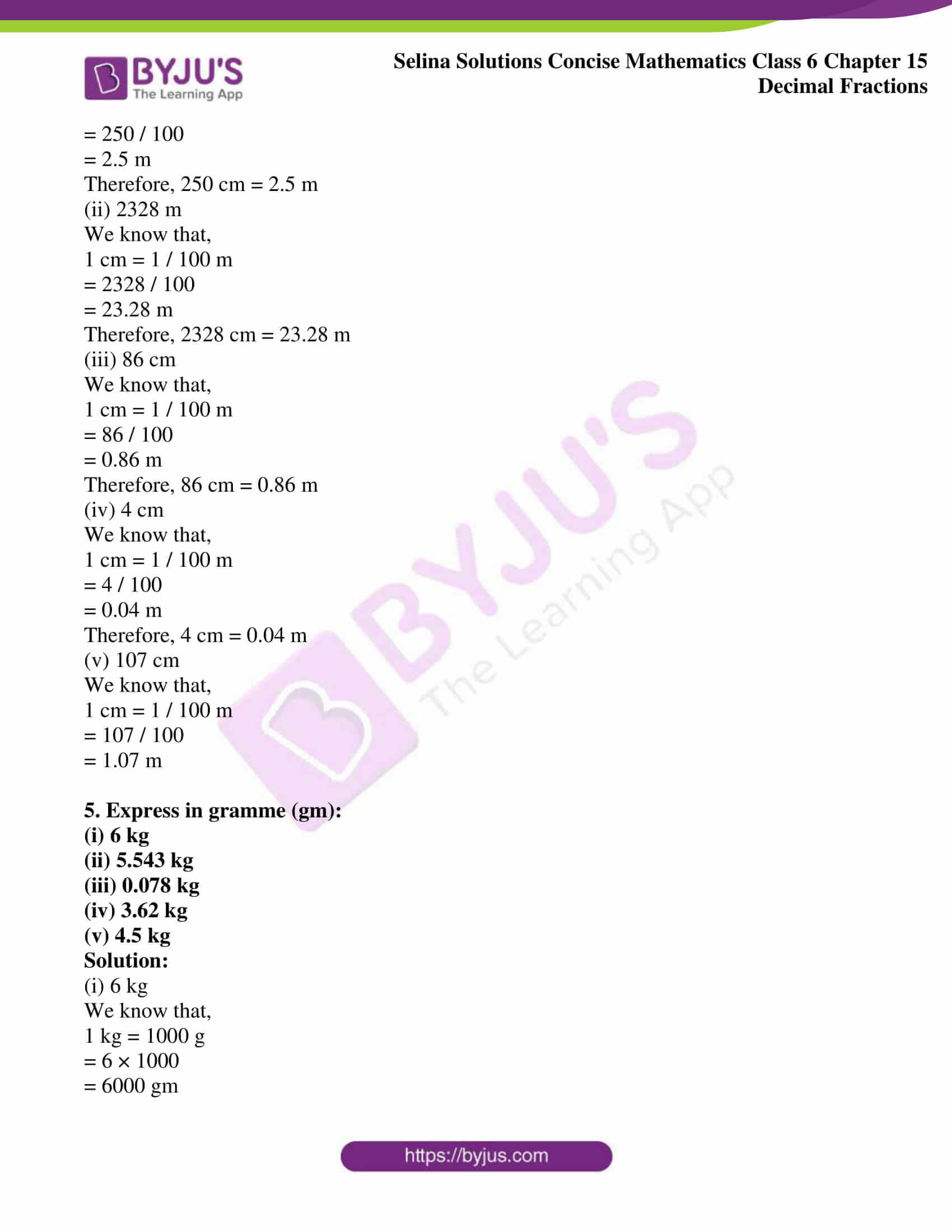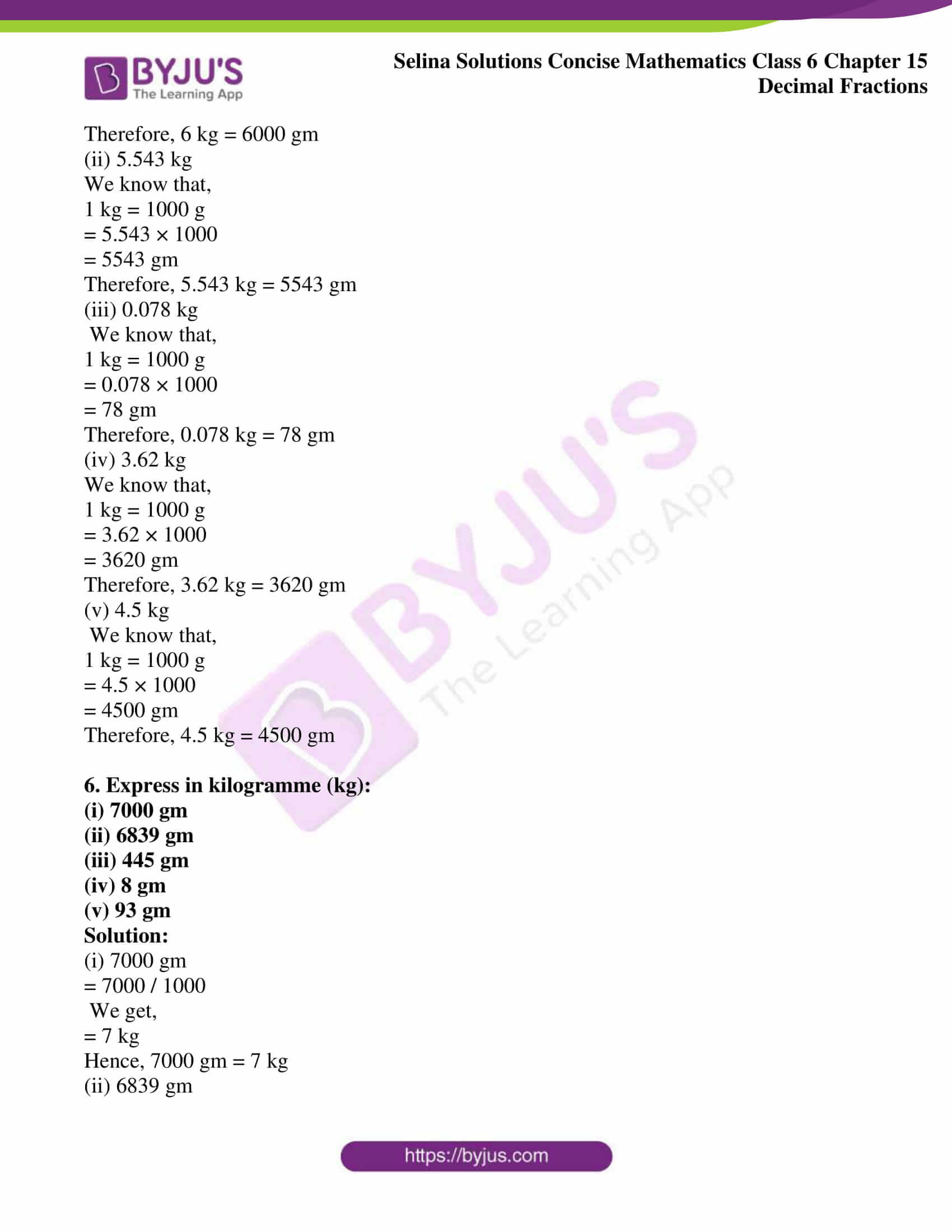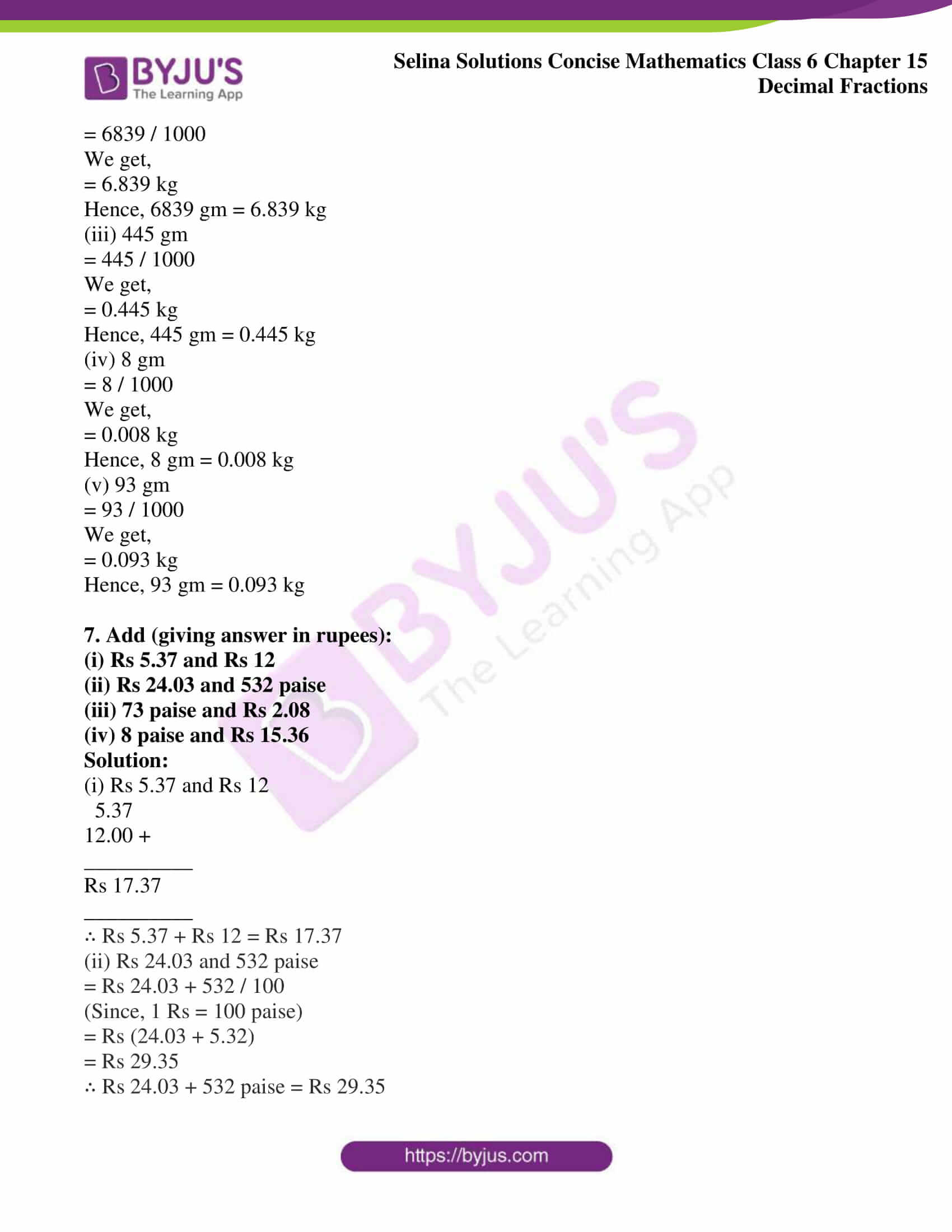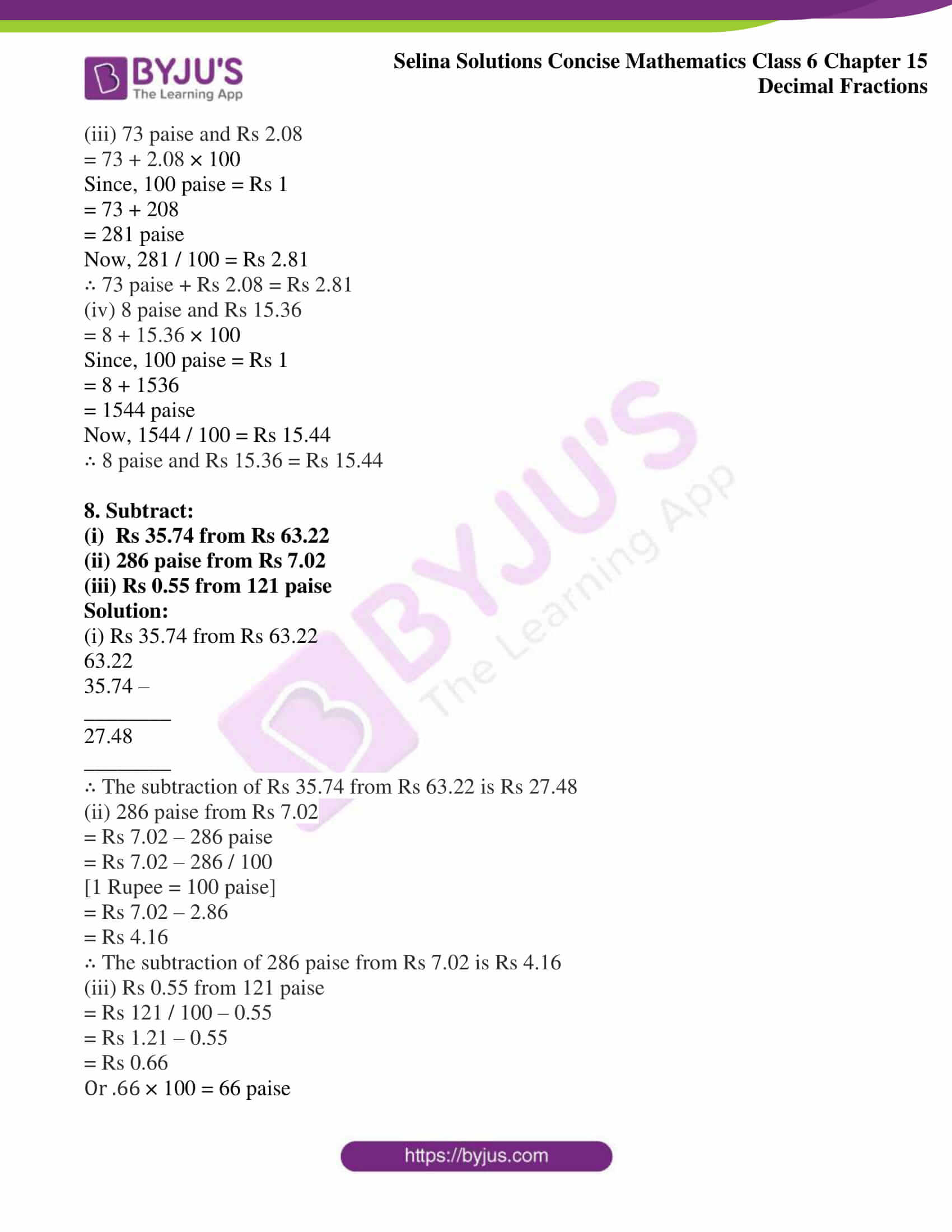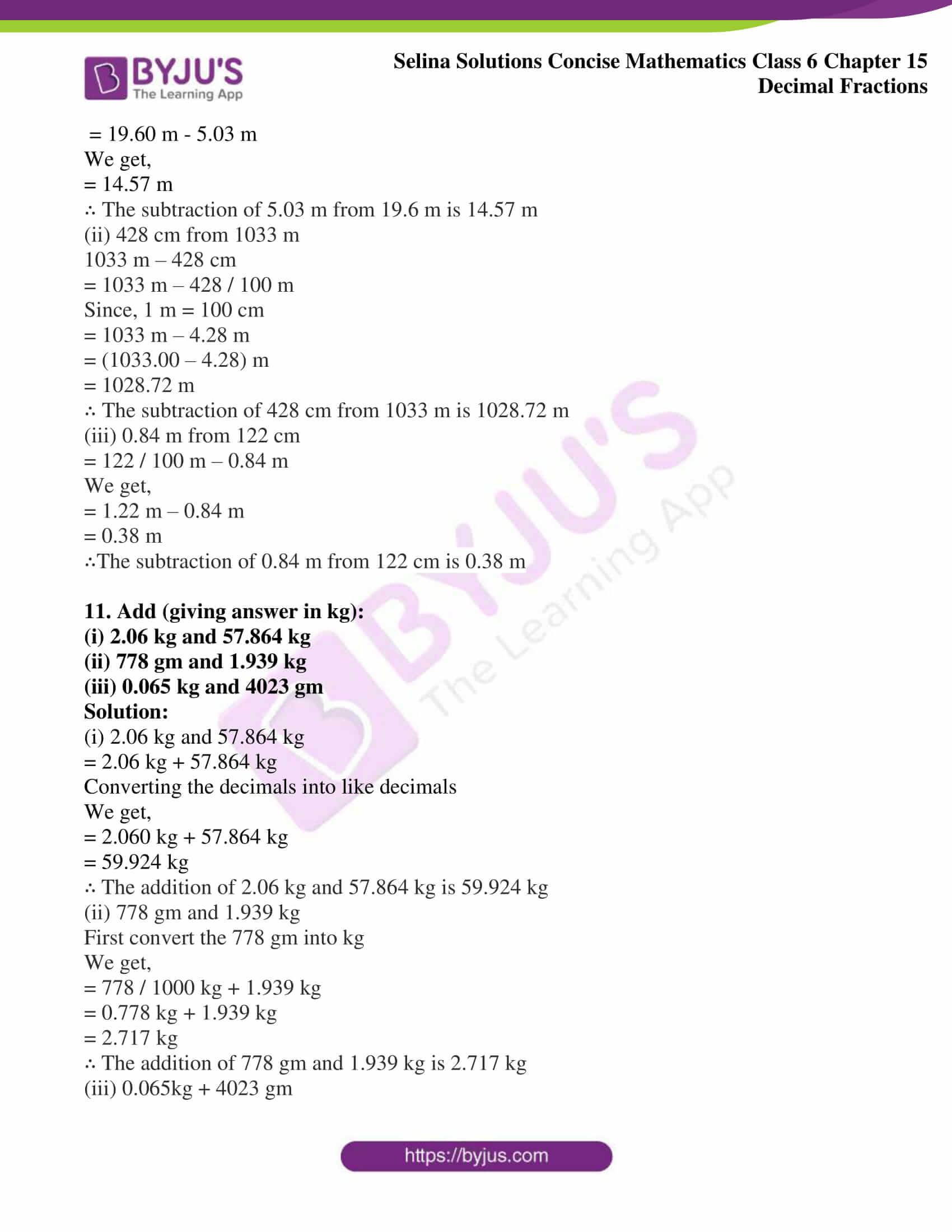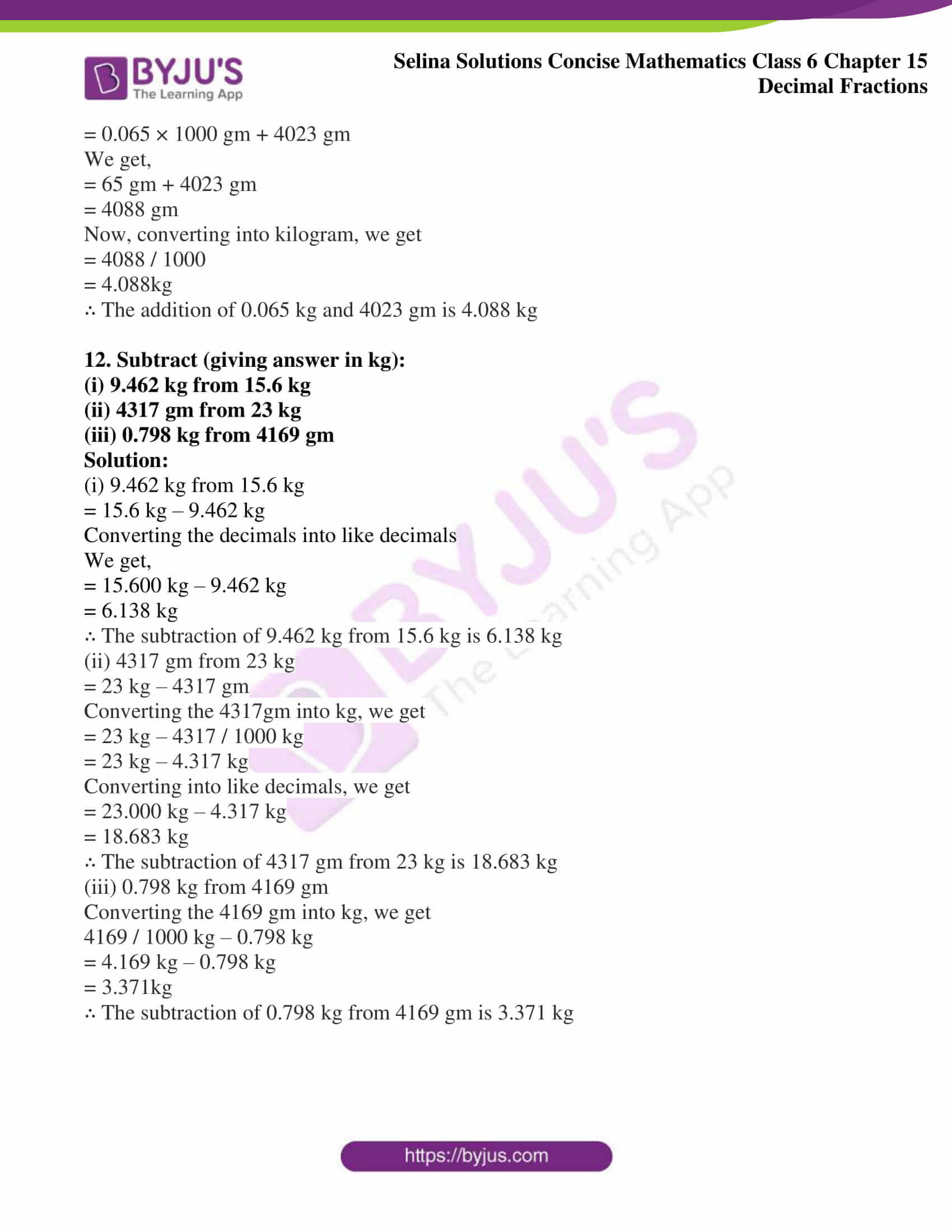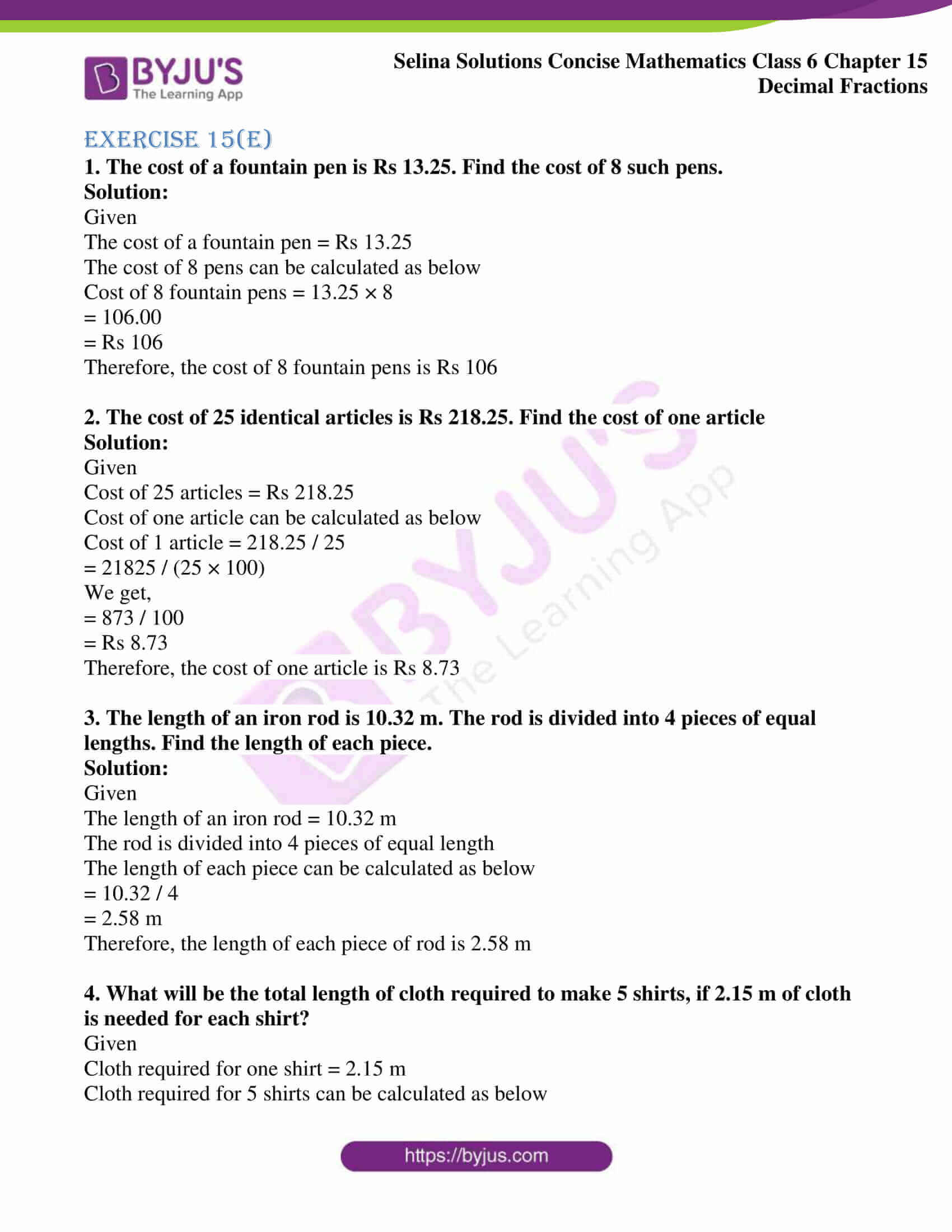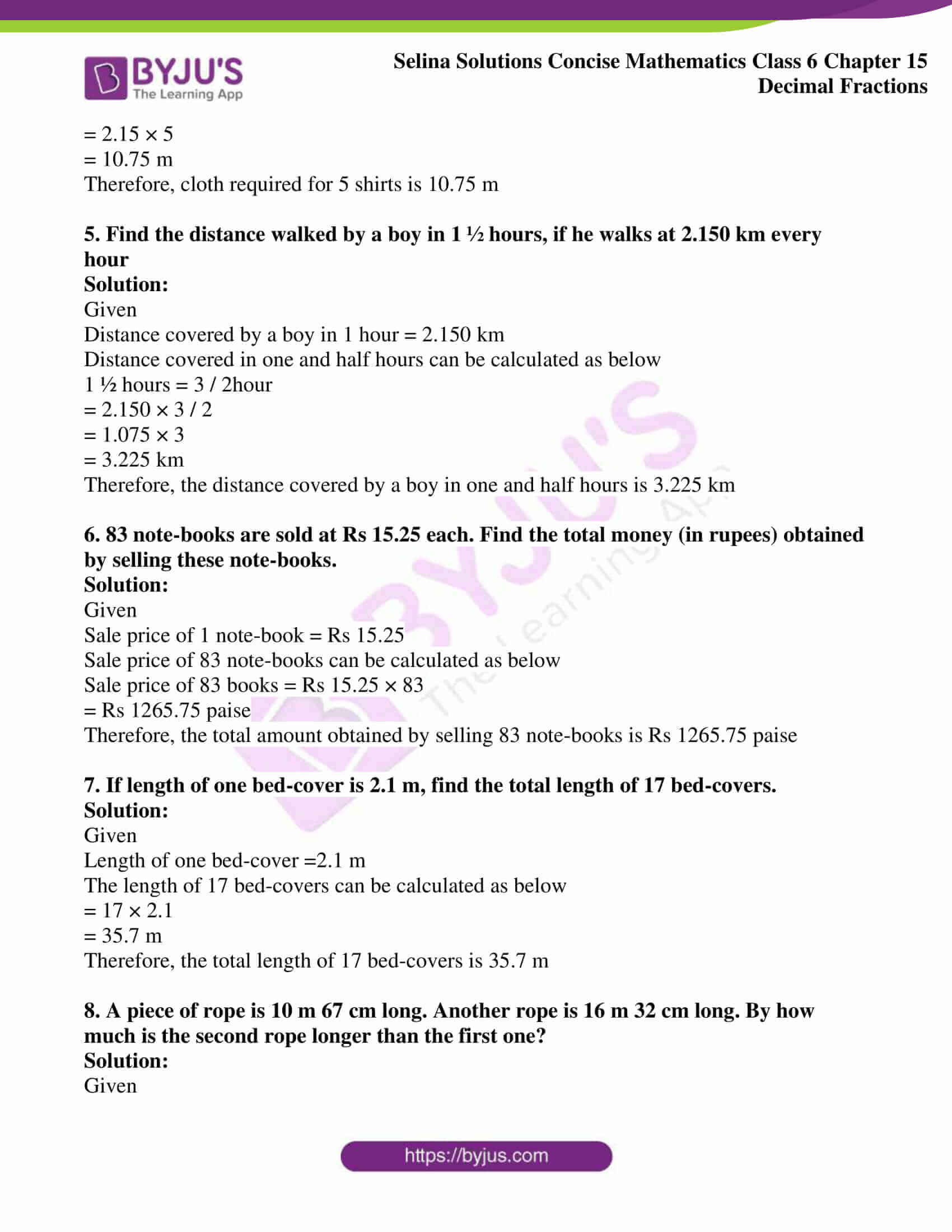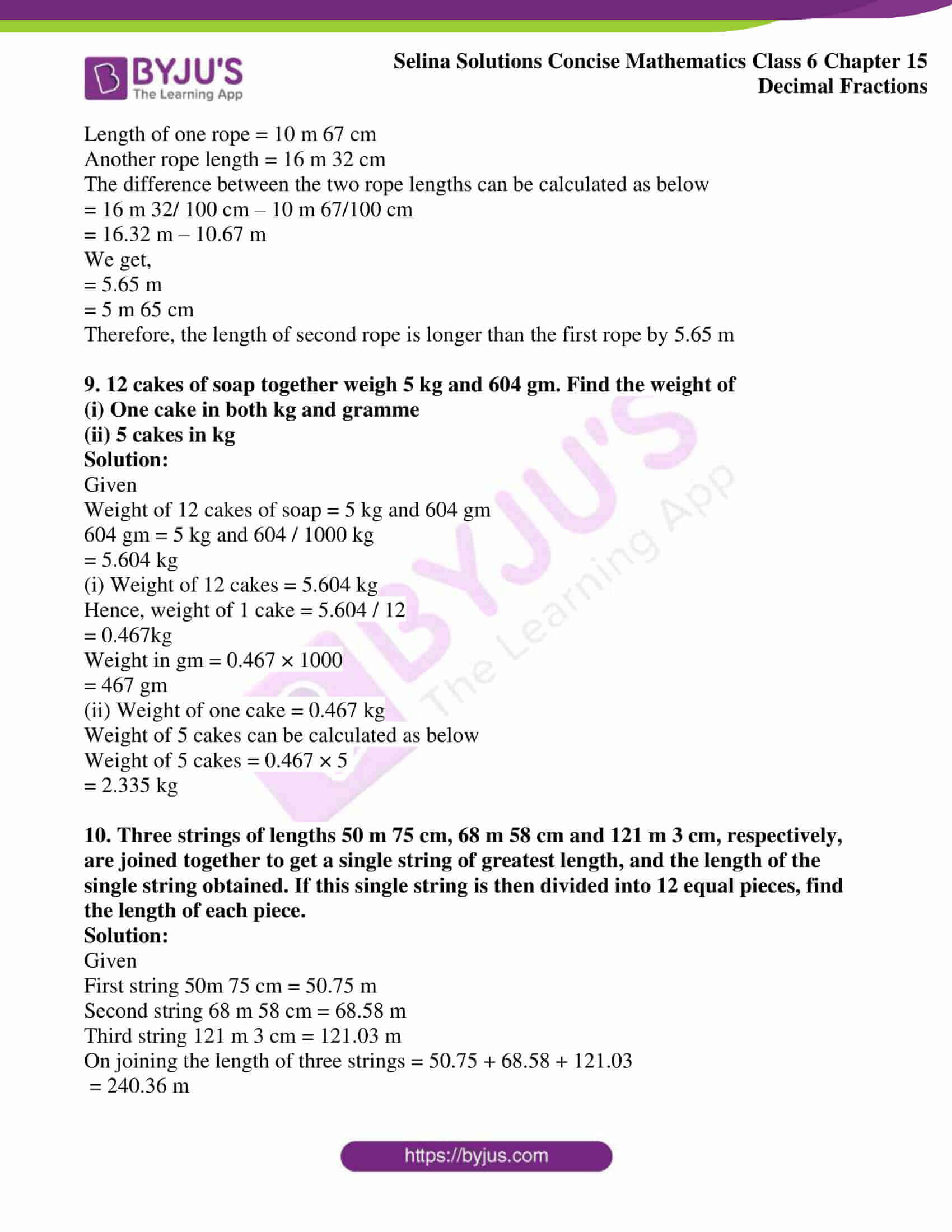### Exercises of Selina Solutions Concise Mathematics Class 6 Chapter 15: Decimal Fractions

Exercise 15(A) Solutions

Exercise 15(B) Solutions

Exercise 15(C) Solutions

Exercise 15(D) Solutions

Exercise 15(E) Solutions

## Access Selina Solutions Concise Mathematics Class 6 Chapter 15: Decimal Fractions

Exercise 15(A)

1. Write the number of decimal places in each of the following:

(i) 7.03

(ii) 0.509

(iii) 146.2

(iv) 0.0065

(v) 8.03207

Solution:

The number of decimal places in a number is the count of digits in its decimal part.

(i) 7.03

The decimal part of the number 7.03 is .03

7.03 has 2 digits in the decimal part

Therefore, 7.03 has 2 decimal places

(ii) 0.509

The decimal part of the number 0.509 is .509

0.509 has 3 digits in the decimal part

Therefore, 0.509 has 3 decimal places

(iii) 146.2

The decimal part of the number 146.2 is .2

146.2 has 1 digit in the decimal part

Therefore, 146.2 has 1 decimal place

(iv) 0.0065

The decimal part of the number 0.0065 is .0065

0.0065 has 4 digits in the decimal part

Therefore, 0.0065 has 4 decimal places

(v) 8.03207

The decimal part of the number 8.03207 is .03207

8.03207 has 5 digits in the decimal part

Therefore, 8.03207 has 5 decimal places

2. Convert the given unlike decimal fractions into like decimal fractions:

(i) 1.36, 239.8 and 47.008

(ii) 507.0752, 8.52073 and 0.808

(iii) 459.22, 7.03093 and 0.200037

Solution:

The like decimal fractions are the numbers that have same number of decimal places. The numbers of decimal places are made equal by adding zeros in the end of number.

(i) 1.36, 239.8 and 47.008

The maximum number of decimal places is in 47.008

It has 3 decimal places

1.36 = 1.360

239.8 = 239. 800

47.008 = 47.008

Hence, the like decimal fractions are 1.360, 239.800 and 47.008

(ii) 507.0752, 8.52073 and 0.808

The maximum number of decimal places is in 8.52073

It has 5 decimal places

507.0752 = 507.07520

8.52073 = 8.52073

0.808 = 0.80800

Hence, the like decimal fractions are 507.07520, 8.52073 and 0.80800

(iii) 459.22, 7.03093 and 0.200037

The maximum number of decimal places is in 0.200037

It has 6 decimal places

459.22 = 459.220000

7.03093 = 7.030930

0.200037 = 0.200037

Hence, the like decimal fractions are 459.220000, 7.030930 and 0.200037

3. Change each of following fractions to a decimal fraction:

(i) 7 / 10

(ii) 47 / 10

(iii) 343 / 100

(iv) 3 / 103

(v) 7295 / 105

Solution:

If a fraction has the numbers like 10, 100, 1000 in its denominator, then to convert into the decimal, the numerator is marked with a decimal point after as many digits from the right as number of zeros in denominator

(i) 7 / 10

To convert into decimal number

Here, number of zeros is 1

Therefore, decimal form of 7 / 10 is 0.7

(ii) 47 / 10

To convert into decimal number

Here, number of zeros is 1

Therefore, decimal form of 47 / 10 is 4.7

(iii) 343 / 100

To convert into decimal number

Here, the number of zeros is 2

Therefore, the decimal form of 343 / 100 is 3.43

(iv) 3 / 103

To convert into decimal number

= 3 / 10 × 10 × 10

= 3 / 1000

Here, number of zeros is 3

Therefore, decimal form of 3 / 103 is 0.003

(v) 7295 / 105

To convert into decimal number

= 7295 / 10 × 10 × 10 × 10 × 10

= 7295 / 100000

Here, number of zeros is 5

Therefore, decimal form of 7295 / 105 is 0.07295

4. Convert into a decimal fraction:

(i) 3 / 4

(ii) 3 / 40

(iii) 1 / 125

(iv) 7 / 25

Solution:

For denominator having no zeros, the denominator and numerator are first multiplied by a factor that makes the number 10 or some power of 10

(i) 3 / 4

To convert into decimal fraction

Multiply and divide the fraction by 25

= (3 × 25) / (4 × 25)

We get,

= 75 / 100

Here, number of zeros is 2

Therefore, the decimal form is 0.75

(ii) 3 / 40

To convert into decimal fraction

Multiply and divide the fraction by 25

= (3 × 25) / (40 × 25)

We get,

= 75 / 1000

Here, number of zeros is 3

Therefore, decimal form is 0.075

(iii) 1 / 125

To convert into decimal fraction

Multiply and divide the fraction by 8

= (1 × 8) / (125 × 8)

We get,

= 8 / 1000

Here, number of zeros is 3

Therefore, decimal form is 0.008

(iv) 7 / 25

To convert into decimal fraction

Multiply and divide the fraction by 4

= (7 × 4) / (25 × 4)

We get,

= 28 / 100

Here, number of zeros is 2

Therefore, decimal form is 0.28

5. Change the given decimals fractions to fraction s in their lowest terms:

(i) 0.05

(ii) 3.95

(iii) 4.005

(iv) 0.876

(v) 50.06

Solution:

(i) 0.05

To convert 0.05 into fraction

Here, the decimal part of the number has 2 digits in the decimal part

Divide the number by 100 and remove the decimal part

We get,

= 5 / 100

= 1 / 20

Therefore, 1 / 20 is the fraction of 0.05

(ii) 3.95

To convert 3.95 into fraction

Here, the decimal part of the number has 2 digits in the decimal part

Divide the number by 100 and remove the decimal

We get,

= 395 / 100

= 79 / 20

=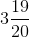Therefore,is the fraction of 3.95

(iii) 4.005

To convert 4.005 into fraction

Here, the decimal part of the number has 3 digits in the decimal part

Divide the number by 1000 and remove the decimal

We get,

= 4005 / 1000

= 801 / 200

=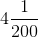Therefore,is the fraction of 4.005

(iv) 0.876

To convert 0.876 into fraction

Here, the decimal part of the number has 3 digits in the decimal part

Divide the number by 1000 and remove the decimal

We get,

= 876 / 1000

= 219 / 250

Therefore, 219 / 250 is the fraction of 0.876

(v) 50.06

To convert 50.06 into fraction

Here, the decimal part of the number has 2 digits in the decimal part

Divide the number by 100 and remove the decimal

We get,

= 5006 / 100

= 2503 / 50

=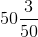Therefore,is the fraction of 50.06

Exercise 15(B)

(i) 0.243, 2.47 and 3.009

(ii) 0.0736, 0.6095 and 0.9107

(iii) 1.01, 257 and 0.200

(iv) 18, 200.35, 11.72 and 2.3

(v) 0.586, 0.0586 and 0.00586

Solution:

To add the decimal number, first convert the decimals into like decimal numbers and then add

(i) 0.243, 2.47 and 3.009

To add 0.243, 2.47 and 3.009,

Convert the decimals into like decimals by adding zeros

Like decimals are 0.243, 2.470 and 3.009

0.243

2.470 +

3.009 +

__________

5.722

___________

Hence, the addition of the given decimal numbers is 5.722

(ii) 0.0736, 0.6095 and 0.9107

To add 0.0736, 0.6095 and 0.9107

Here, all the numbers are like decimal numbers

0.0736

0.6095 +

0.9107 +

___________

1.5938

___________

Hence, the addition of the given numbers is 1.5938

(iii) 1.01, 257 and 0.200

To add 1.01, 257 and 0.200

Convert the decimals into like decimal by adding zeros

Like decimals are 1.010, 257.000 and 0.200

1.010

257.000 +

0.200 +

____________

258.210

____________

Hence, the addition of the given decimal numbers is 258.210

(iv) 18, 200.35, 11.72 and 2.3

To add 18, 200.35, 11.72 and 2.3

Convert the decimals into like decimals by adding zeros

Like decimals are 18.00, 200.35, 11.72 and 2.30

18.00

200.35 +

11.72 +

2.30 +

____________

232.37

____________

Hence, the addition of the given decimal numbers is 232.37

(v) 0.586, 0.0586 and 0.00586

To add 0.586, 0.0586 and 0.00586

Convert the decimals into like decimals by adding zeros

Like decimals are 0.58600, 0.05860 and 0.00586

0.58600

0.05860 +

0.00586 +

___________

0.65046

___________

Hence, the addition of the given decimal numbers is 0.65046

2. Find the value of:

(i) 6.8 – 2. 64

(ii) 2 – 1.0304

(iii) 0.1 – 0.08

(iv) 0.83 – 0.342

Solution:

To subtract the decimal numbers, first convert the decimal into like decimal numbers and then subtract

(i) 6.8 – 2. 64

Convert the decimals into like decimal numbers by adding zeros

Like decimals are 6.80 and 2.64

6.80 –

2.64

________

4.16

_________

Hence, the subtraction of the decimal numbers is 4.16

(ii) 2 – 1.0304

Convert the decimals into like decimal numbers by adding zeros

Like decimals are 2.0000 and 1.0304

2.0000 –

1.0304

__________

0.9696

___________

Hence, the subtraction of the decimal numbers is 0.9696

(iii) 0.1 – 0.08

Convert the decimals into like decimal numbers by adding zeros

Like decimals are 0.10 and 0.08

0.10

0.08 –

_________

0.02

_________

Hence, the subtraction of the decimal numbers is 0.02

(iv) 0.83 – 0.342

Convert the decimals into like decimal numbers by adding zeros

Like decimals are 0.830 and 0.342

0.830

0.342 –

__________

0.488

___________

Hence, the subtraction of the decimal numbers is 0.488

3. Subtract:

(i) 0.43 from 0.97

(ii) 2.008 from 22.1058

(iii) 0.18 from 0.6

(iv) 1.002 from 17

(v) 83 from 92.05

Solution:

To subtract the decimal numbers, first convert the decimals into like decimal numbers and then subtract

(i) 0.43 from 0.97

The given decimals are like decimals

0.97

0.43 –

________

0.54

_________

Hence, the subtraction of the decimal numbers is 0.54

(ii) 2.008 from 22.1058

To subtract 2.008 from 22.1058

Convert the decimals into like decimals by adding zeros

Like decimals are 22.1058 and 2.0080

22.1058

2.0080 –

_________

20.0978

__________

Hence, the subtraction of the decimal numbers is 20.0978

(iii) 0.18 from 0.6

To subtract 0.18 from 0.6

Convert the decimals into like decimals by adding zeros

Like decimals are 0.60 and 0.18

0.60

0.18 –

_________

0.42

__________

Hence, the subtraction of the decimal numbers is 0.42

(iv) 1.002 from 17

To subtract 1.002 from 17

Convert the decimals into like decimals by adding zeros

Like decimals are 17.000 and 1.002

17.000

1.002 –

_________

15.998

__________

Hence, the subtraction of the decimal numbers is 15.998

(v) 83 from 92.05

To subtract 83 from 92.05

Convert the decimals into like decimals by adding zeros

Like decimals are 92.05 and 83.00

92.05

83.00 –

__________

9.05

___________

Hence, the subtraction of the decimal numbers is 9.05

4. Simplify:

(i) 3.5 – 2.43 + 0.075

(ii) 7.84 + 0.3 – 4.016

(iii) 2.987 – 1.25 – 0.54

(iv) 52.9 – 231.666 + 204

(v) 8.57 – 6.4432 – 1.70 + 0.683

Solution:

To simplify the expression, first convert the decimal numbers into like decimals and perform addition and subtraction

(i) 3.5 – 2.43 + 0.075

To simplify 3.5 – 2.43 + 0.075

Convert the decimals into like decimal numbers

Like decimals are 3.500, 2.430 and 0.075

= 3.500 – 2.430 + 0.075

= 3.500 + 0.075 – 2.430

= 3.575 – 2.430

= 1.145

Therefore, the simplification of the given expression is 1.145

(ii) 7.84 + 0.3 – 4.016

To simplify 7.84 + 0.3 – 4.016

Convert the decimals into like decimal numbers

Like decimals are 7.840, 0.300 and 4.016

= 7.840 + 0.300 – 4.016

= 8.140 – 4.016

= 4.124

Therefore, the simplification of the given expression is 4.124

(iii) 2.987 – 1.25 – 0.54

To simplify 2.987 – 1.25 – 0.54

Convert the decimals into like decimal numbers

Like decimals are 2.987, 1.250 and 0.540

= 2.987 – 1.250 – 0.540

= 1.737 – 0.540

= 1.197

Therefore, the simplification of the given expression is 1.197

(iv) 52.9 – 231.666 + 204

To simplify 52.9 – 231.666 + 204

Convert the decimals into like decimal numbers

Like decimals are 52.900, 231.666 and 204.000

= 52.900 – 231.666 + 204.000

= 256.900 – 231.666

= 25.234

Therefore, the simplification of the given expression is 25.234

(v) 8.57 – 6.4432 – 1.70 + 0.683

To simplify 8.57 – 6.4432 – 1.70 + 0.683

Convert the decimals into like decimal numbers

Like decimals are 8.5700, 6.4432, 1.7000 and 0.6830

= 8.5700 – 6.4432 – 1.7000 + 0.6830

= 9.253 – 8.1432

= 1.1098

Therefore, the simplification of the given expression is 1.1098

5. From the sum of 75.75 and 4.9 subtract 28.465

Solution:

To subtract 28.465 from the sum of 75.75 and 4.9

Convert the numbers into like decimal numbers

Like decimals are 75.75 and 4.90

75.75

4.90 +

___________

80.65

____________

Now, to subtract 28.465 from 80.65

Convert the numbers into like decimal numbers

Like decimals are 28.465 and 80.650

80.650

28.465 –

___________

52.185

____________

Hence, the simplification of the sum and subtraction is 52.185

6. Subtract the sum of 8.14 and 12.9 from 32.7

Solution:

To subtract the sum of 8.14 and 12.9 from 32.7

Convert the numbers into like decimal numbers

Like decimals are 8.14 and 12.90

8.14

12.90 +

____________

21.04

_____________

To subtract 21.04 from 32.7

Convert the numbers into like decimal numbers

Like decimals are 21.04 and 32.70

32.70

21.04 –

___________

11.66

____________

Therefore, the simplification of the sum and subtraction is 11.66

7. Subtract the sum of 34.27 and 159.8 from the sum of 20.937 and 200.6

Solution:

To subtract the sum of 34.27 and 159.8 from the sum of 20.937 and 200.6

Convert the numbers into like decimal numbers

Like decimals are 34.27 and 159.80

34.27

159.80 +

____________

194.07

____________

To add 20.937 and 200.6 convert the numbers into like decimal numbers

Like decimals are 20.937 an 200.600

20.937

200.600 +

____________

221.537

_____________

To subtract 194.07 from 221.537

Convert the numbers into like decimal numbers

221.537

194.070 –

____________

27.467

_____________

Hence, the subtraction of above decimal numbers is 27.467

8. From the sum of 2.43 and 4.349 subtract the sum of 0.8 and 3.15

Solution:

To subtract the sum of 0.8 and 3.15 from sum of 2.43 and 4.349

Convert the numbers into like decimal numbers

Like decimals are 0.80 and 3.15

0.80

3.15 +

_________

3.95

__________

Convert the numbers into like decimal numbers

2.430

4.349 +

___________

6.779

____________

To subtract 3.95 from 6.779

Convert the numbers into like decimal numbers

6.779

3.950 –

_______________

2.829

________________

Therefore, the subtraction of above decimal numbers is 2.829

9. By how much does the sum of 18.0495 and 34.9644 exceed the sum of 7.6752 and 24.876?

Solution:

To obtain the difference between the sum of 18.0495 and 34.9644 and the sum of 7.6752 and 24.876

Now, to add 18.0495 and 34.9644

Convert the numbers into like decimal numbers

The given decimals are like decimals

18.0495

34.9644 +

____________

53.0139

____________

Now, to add 7.6752 and 24.876

Convert the numbers into like decimal numbers

Like decimals are 7.6752 and 24.8760

7.6752

24.8760 +

__________

32.5512

___________

To subtract 32.5512 from 53.0139

Convert the numbers into like decimal numbers

The given decimals are like decimals

53.0139

32.5512 –

__________

20.4627

___________

Hence, the sum exceed the other decimal numbers by 20.4627

10. What least number must be added to 89.376 to get 1000?

Solution:

The number to be added to 89.376 to get 1000

Subtract 89.376 from 1000

Convert the decimals into like decimal numbers

Like decimals are 89.376 and 1000.000

1000.000

89.376 –

__________

910.624

___________

Therefore, the number to be added to 89.376 to obtain 1000 is 910.624

Exercise 15(C)

1. Multiply:

(i) 5.6 and 8

(ii) 38.46 and 9

(iii) 0.943 and 62

(iv) 0.0453 and 35

(v) 7.5 and 2.5

Solution:

(i) 5.6 and 8

The multiplication of 5.6 and 8 is as follows

5.6 × 8 = 44.8

Hence, 5.6 × 8 = 44.8

(ii) 38.46 and 9

The multiplication of 38.46 and 9 is as follows

38.46 × 9 = 346.14

Hence, 38.46 × 9 = 346.14

(iii) 0.943 and 62

The multiplication of 0.943 and 62 is as follows

943

62 ×

________

1886

5658×

________

58466

________

We know that,

.943 × 62 = 58.466

Hence 0.943 × 62 = 58.466

(iv) 0.0453 and 35

The multiplication of 0.0453 and 35 is as follows

453

35×

_________

2265

1359×

__________

15855

___________

We know that,

453 × 35 = 15855

Hence 0.0453 × 35 = 1.5855

(v) 7.5 and 2.5

The multiplication of 7.5 and 2.5 is as follows

75

25 ×

________

375

150×

_________

1875

_________

We know that,

75 × 25 = 1875

Hence 7.5 × 2.5 = 18.75

2. Evaluate:

(i) 0.0008 × 26

(ii) 0.038 × 95

(iii) 1.2 × 2.4 × 3.6

(iv) 0.9 × 1.8 × 0.27

(v) 1.5 × 1.5 × 1.5

Solution:

(i) 0.0008 × 26

Since,

8 × 26 =208

0.0008 × 26 = 0.0208

∴ We get 0.0208 on multiplying 0.0008 × 26

(ii) 0.038 × 95

38

95×

_______

190

342×

________

3610

________

Since,

38 × 95 = 3610

.038 × 95 = 3.610

= 3.61

∴ We get 3.61 on multiplying 0.038 × 95

(iii) 1.2 × 2.4 × 3.6

12

24 ×

_______

48

24×

_________

288

36 ×

__________

1728

864×

_________

10368

_________

Since,

12 × 24 × 36 = 10368

1.2 × 2.4 × 3.6 = 10.368

∴ We get 10.368 on multiplying 1.2 × 2.4 × 3.6

(iv) 0.9 × 1.8 × 0.27

9

18 ×

_______

72

_________

162

27 ×

________

1134

324 ×

_________

4374

_________

Since,

9 × 18 × 27 = 4374

0.9 × 1.8 × 0.27 = 0.4374

∴ We get 0.4374 on multiplying 0.9 × 1.8 × 0.27

(v) 1.5 × 1.5 × 1.5

15

15 ×

________

75

15 ×

_________

225

15 ×

__________

1125

225 ×

__________

3375

___________

Since,

15 × 15 × 15 = 3375

1.5 × 1.5 × 1.5 = 3.375

3. Multiply each of the following numbers by 10, 100 and 1000:

(i) 3.9

(ii) 2.89

(iii) 0.0829

(iv) 40.3

(v) 0.3725

Solution:

(i) 3.9

3.9 × 10 = 39

3.9 × 100 = 390

3.9 × 1000 = 3900

Hence, 39, 390 and 3900 are the required numbers

(ii) 2.89

2.89 × 10 = 28.9

2.89 × 100 = 289

2.89 × 1000 = 2890.00

= 2890

Hence, 28.9, 289 and 2890 are the required numbers

(iii) 0.0829

0.0829 × 10 = 0.829

0.0829 × 100 = 8.29

0.0829 × 1000 = 82.9

Hence, 0.829, 8.29 and 82.9 are the required numbers

(iv) 40.3

40.3 × 10 = 403

40.3 × 100 = 4030

40.3 × 1000 = 40300

Hence, 403, 4030 and 40300 are the required numbers

(v) 0.3725

0.3725 × 10 = 3.725

0.3725 × 100 = 37.25

0.3725 × 1000 = 372.5

4. Evaluate:

(i) 8.64 ÷ 8

(ii) 0.0072 ÷ 6

(iii) 20.64 ÷ 16

(iv) 1.602 ÷ 15

(v) 13.08 ÷ 4

Solution:

(i) 8.64 ÷ 8

8.64 ÷ 8 = 8.64 / 8

We get

= 1.08

Therefore, the value of 8.64 ÷ 8 = 1.08

(ii) 0.0072 ÷ 6

0.0072 ÷ 6 = (0.0072) / 6

We get

= 0.0012

Therefore, the value of 0.0072 ÷ 6 = 0.0012

(iii) 20.64 ÷ 16

20.64 ÷ 16 = (20.64) / 16

We get

= 1.29

Therefore, the value of 20.64 ÷ 16 = 1.29

(iv) 1.602 ÷ 15

1.602 ÷ 15 = (1.602) / 15

We get

1602 / (1000 × 15)

We get

= 106.8 / 1000

= 0.1068

Therefore, the value of 1.602 ÷ 15 = 0.1068

(v) 13.08 ÷ 4

13.08 ÷ 4 = 13.08 / 4

We get

= 3.27

Therefore, the value of 13.08 ÷ 4 = 3.27

5. Divide each of the following numbers by 10, 100 and 1000:

(i) 49.79

(ii) 0.923

(iii) 0.0704

Solution:

(i) 49.79

49.79 / 10 = 4.979

49.79 / 100 = 0.4979

49.79 / 1000 = 0.04979

Therefore, the required numbers are 4.979, 0.4979 and 0.04979

(ii) 0.923

0.923 / 10 = 0.0923

0.923 / 100 = 0.00923

0.923 / 1000 = 0.000923

Therefore, the required numbers are 0.0923, 0.00923 and 0.000923

(iii) 0.0704

0.0704 / 10 = 0.00704

0.0704 / 100 = 0.000704

0.0704 / 1000 = 0.0000704

Therefore, the required numbers are 0.00704, 0.000704 and 0.0000704

6. Evaluate:

(i) 9.4 ÷ 0.47

(ii) 6.3 ÷ 0.09

(iii) 2.88 ÷ 1.2

(iv) 8.64 ÷ 1.6

(v) 37.188 ÷ 3.6

Solutions:

(i) 9.4 ÷ 0.47

= 9.4 / 0.47

= (94 × 100) / (47 × 10)

On calculating further, we get

= 2 × 10

= 20

Hence, 9.4 ÷ 0.47 = 20

(ii) 6.3 ÷ 0.09

= 6.3 / 0.09

= (63 × 100) / (9 × 10)

We get

= 6300 / 90

= 630 / 9

= 70

Hence, 6.3 ÷ 0.09 = 70

(iii) 2.88 ÷ 1.2

= 2.88 / 1.2

= (288 × 10) / (12 × 100)

We get,

= 2880 / 1200

= 288 / 120

= 2.4

Hence, 2.88 ÷ 1.2 = 2.4

(iv) 8.64 ÷ 1.6

= 8.64 / 1.6

= (8.64 × 10) / (1.6 × 10)

We get,

= 86.4 / 16

= 5.4

Hence, 8.64 ÷ 1.6 = 5.4

(v) 37.188 ÷ 3.6

= 37.188 / 3.6

= (37188 × 10) / (36 × 1000)

We get,

= 371880 / 36000

= 2066 / 200

= 1033 / 100

= 10.33

7. Fill in the blanks with 10, 100, 1000, or 10000 etc:

(i) 7.85 × ……… = 78.5

(ii) 0.442 × ………. = 442

(iii) 0.0924 × ………. = 9.24

(iv) 0.00187 × ……… = 18.7

(v) 2.6 × …….. = 2600

Solution:

(i) 7.85 × 10 = 78.5

(ii) 0.442 × 1000 = 442

(iii) 0.0924 × 100 = 9.24

(iv) 0.00187 × 10000 = 18.7

(v) 2.6 × 1000 = 2600

8. Evaluate:

(i) 9.32 – 28.54 ÷ 10

(ii) 0.234 × 10 + 62.8

(iii) 3.06 × 100 – 889.4 ÷ 100

(iv) 2.86 × 7.5 + 45.4 ÷ 0.2

(v) 97. 82 × 0.03 – 0.54 ÷ 0.3

Solution:

(i) 9.32 – 28.54 ÷ 10

= 9.32 – 2.854

So, we get

= 9.320 – 2.854

= 6.466

Therefore, 9.32 – 28.54 ÷ 10 = 6.466

(ii) 0.234 × 10 + 62.8

Using BODMAS, we get

= 2.34 + 62.80

= 65.14

Therefore, 0.234 × 10 + 62.8 = 65.14

(iii) 3.06 × 100 – 889.4 ÷ 100

Using BODMAS, we get

= 3.06 × 100 – 8.894

= 306 – 8.894

= 306.000 – 8.894

= 297.106

(iv) 2.86 × 7.5 + 45.4 ÷ 0.2

Using BODMAS, we get

= 2.86 × 7.5 + 454 ÷ 2

On further calculation, we get

= 2.86 × 7.5 + 227.00

= (286 / 100) × (75 / 10) + 227.00

= (286 / 4) × (3 / 10) + 227.00

= (143 / 2) × (3 / 10) + 227.00

We get,

= 429 / 20 + 227.00

= 21.45 + 227.00

= 248.45

(v) 97. 82 × 0.03 – 0.54 ÷ 0.3

= 97.82 × 0.03 – 0.54 / 0.3

= 97.82 × 0.03 – (0.54 × 10) / (0.3 × 10)

On further calculation, we get

= 2.9346 – 5.4 / 3

= 2.9346 – 1.8

= 2.9346 – 1.8000

We get,

= 1.1346

Exercise 15(D)

1. Express in paise:

(i) Rs 8.40

(ii) Rs 0.97

(iii) Rs 0.09

(iv) Rs 62.35

Solution:

(i) Rs 8.40

= 8.40 × 100 paise [WKT 1Rs = 100 Paise]

= (840 / 100) × 100 Paise

On substituting, we get

= 840 Paise

Therefore, Rs 8.40 = 840 paise

(ii) Rs 0.97

= 0.97 × 100 paise [WKT 1 Rs = 100 Paise]

On calculating, we get

= 97 paise

Therefore, Rs 0.97 = 97 paise

(iii) Rs 0.09

= 0.09 × 100 [WKT 1 Rs = 100 Paise]

We get,

= 9.00 paise

Therefore, Rs 0.09 = 9.00 paise

(iv) Rs 62.35

= 62.35 × 100 paise [WKT 1 Rs = 100 Paise]

= (6235 / 100) × 100 paise

We get

= 6235 paise

Therefore, Rs 62.35 = 6235 paise

2. Express in rupees:

(i) 55 P

(ii) 8 P

(iii) 695 P

(iv) 3279 P

Solution:

(i) 55 P

We know that,

1 P = 1 / 100 Rs

= 55 / 100

We get,

= Rs 0.55

Hence, the expression for 55 P in the form of rupees is Rs 0.55

(ii) 8 P

We know that,

1 P = 1 / 100 Rs

= 8 / 100

We get,

= Rs 0.08

Hence, the expression for 8 P in the form of rupees is Rs 0.08

(iii) 695 P

We know that,

1 P = 1 / 100 Rs

= 695 / 100

We get,

= Rs 6.95

Hence, the expression for 695 P in the form of rupees is Rs 6.95

(iv) 3279 P

We know that,

1 P = 1 / 100 Rs

= 3279 / 100

We get,

= Rs 32.79

Hence, the expression for 3279 P in the form of rupees is Rs 32.79

3. Express in centimetre (cm):

(i) 6 m

(ii) 8.54 m

(iii) 3.08 m

(iv) 0.87 m

(v) 0.03 m

Solution:

(i) 6 m

We know that 1 m = 100 cm

= 6 × 100

We get,

= 600 cm

Hence, 6 m = 600 cm

(ii) 8.54 m

We know that,

1 m = 100 cm

= 8.54 × 100

We get,

= 854 cm

Hence, 8.54 m = 854 cm

(iii) 3.08

We know that,

1 m = 100 cm

= 3.08 × 100

We get,

= 308 cm

Hence, 3.08 m = 308 cm

(iv) 0.87 m

We know that,

1 m = 100 cm

= 0.87 × 100

We get,

= 87 cm

Hence, 0.87 m = 87 cm

(v) 0.03 m

We know that,

1 m = 100 cm

= 0.03 × 100

We get,

= 3 cm

Hence, 0.03 m = 3 cm

4. Express in metre (m):

(i) 250 cm

(ii) 2328 cm

(iii) 86 cm

(iv) 4 cm

(v) 107 cm

Solution:

(i) 250 cm

We know that,

1 cm = 1 / 100 m

= 250 / 100

= 2.5 m

Therefore, 250 cm = 2.5 m

(ii) 2328 m

We know that,

1 cm = 1 / 100 m

= 2328 / 100

= 23.28 m

Therefore, 2328 cm = 23.28 m

(iii) 86 cm

We know that,

1 cm = 1 / 100 m

= 86 / 100

= 0.86 m

Therefore, 86 cm = 0.86 m

(iv) 4 cm

We know that,

1 cm = 1 / 100 m

= 4 / 100

= 0.04 m

Therefore, 4 cm = 0.04 m

(v) 107 cm

We know that,

1 cm = 1 / 100 m

= 107 / 100

= 1.07 m

5. Express in gramme (gm):

(i) 6 kg

(ii) 5.543 kg

(iii) 0.078 kg

(iv) 3.62 kg

(v) 4.5 kg

Solution:

(i) 6 kg

We know that,

1 kg = 1000 g

= 6 × 1000

= 6000 gm

Therefore, 6 kg = 6000 gm

(ii) 5.543 kg

We know that,

1 kg = 1000 g

= 5.543 × 1000

= 5543 gm

Therefore, 5.543 kg = 5543 gm

(iii) 0.078 kg

We know that,

1 kg = 1000 g

= 0.078 × 1000

= 78 gm

Therefore, 0.078 kg = 78 gm

(iv) 3.62 kg

We know that,

1 kg = 1000 g

= 3.62 × 1000

= 3620 gm

Therefore, 3.62 kg = 3620 gm

(v) 4.5 kg

We know that,

1 kg = 1000 g

= 4.5 × 1000

= 4500 gm

Therefore, 4.5 kg = 4500 gm

6. Express in kilogramme (kg):

(i) 7000 gm

(ii) 6839 gm

(iii) 445 gm

(iv) 8 gm

(v) 93 gm

Solution:

(i) 7000 gm

= 7000 / 1000

We get,

= 7 kg

Hence, 7000 gm = 7 kg

(ii) 6839 gm

= 6839 / 1000

We get,

= 6.839 kg

Hence, 6839 gm = 6.839 kg

(iii) 445 gm

= 445 / 1000

We get,

= 0.445 kg

Hence, 445 gm = 0.445 kg

(iv) 8 gm

= 8 / 1000

We get,

= 0.008 kg

Hence, 8 gm = 0.008 kg

(v) 93 gm

= 93 / 1000

We get,

= 0.093 kg

Hence, 93 gm = 0.093 kg

(i) Rs 5.37 and Rs 12

(ii) Rs 24.03 and 532 paise

(iii) 73 paise and Rs 2.08

(iv) 8 paise and Rs 15.36

Solution:

(i) Rs 5.37 and Rs 12

5.37

12.00 +

__________

Rs 17.37

__________

∴ Rs 5.37 + Rs 12 = Rs 17.37

(ii) Rs 24.03 and 532 paise

= Rs 24.03 + 532 / 100

(Since, 1 Rs = 100 paise)

= Rs (24.03 + 5.32)

= Rs 29.35

∴ Rs 24.03 + 532 paise = Rs 29.35

(iii) 73 paise and Rs 2.08

= 73 + 2.08 × 100

Since, 100 paise = Rs 1

= 73 + 208

= 281 paise

Now, 281 / 100 = Rs 2.81

∴ 73 paise + Rs 2.08 = Rs 2.81

(iv) 8 paise and Rs 15.36

= 8 + 15.36 × 100

Since, 100 paise = Rs 1

= 8 + 1536

= 1544 paise

Now, 1544 / 100 = Rs 15.44

∴ 8 paise and Rs 15.36 = Rs 15.44

8. Subtract:

(i) Rs 35.74 from Rs 63.22

(ii) 286 paise from Rs 7.02

(iii) Rs 0.55 from 121 paise

Solution:

(i) Rs 35.74 from Rs 63.22

63.22

35.74 –

________

27.48

________

∴ The subtraction of Rs 35.74 from Rs 63.22 is Rs 27.48

(ii) 286 paise from Rs 7.02

= Rs 7.02 – 286 paise

= Rs 7.02 – 286 / 100

[1 Rupee = 100 paise]

= Rs 7.02 – 2.86

= Rs 4.16

∴ The subtraction of 286 paise from Rs 7.02 is Rs 4.16

(iii) Rs 0.55 from 121 paise

= Rs 121 / 100 – 0.55

= Rs 1.21 – 0.55

= Rs 0.66

Or .66 × 100 = 66 paise

∴ The subtraction of Rs 0.55 from 121 paise = 66 paise

(i) 2.4 m and 1.78 m

(ii) 848 cm and 2.9 m

(iii) 0.93 m and 64 cm

Solution:

(i) 2.4 m and 1.78 m

2.40 m

1.78 m +

_________

4.18 m

_________

∴ The addition of 2.4 m and 1.78 m = 4.18 m

(ii) 848 cm and 2.9 m

= 848 / 100 m + 2.9 m {1 m = 100 cm}

= 8.48 m + 2.9 m

8.48

2.9 +

___________

11.38

____________

∴ The addition of 848 cm and 2.9 m = 11.38 m

(iii) 0.93 m + 64 cm

= 0.93 m + 64 / 100 cm

= 0.93 m + 0.64 m

0.93

0.64 +

___________

1.57

____________

∴ The addition of 0.93 m + 64 cm = 1.57 m

10. Subtract (giving answer in metre):

(i) 5.03 m from 19.6 m

(ii) 428 cm from 1033 m

(iii) 0.84 m from 122 cm

Solution:

(i) 5.03 m from 19.6 m

= 19.60 m – 5.03 m

We get,

= 14.57 m

∴ The subtraction of 5.03 m from 19.6 m is 14.57 m

(ii) 428 cm from 1033 m

1033 m – 428 cm

= 1033 m – 428 / 100 m

Since, 1 m = 100 cm

= 1033 m – 4.28 m

= (1033.00 – 4.28) m

= 1028.72 m

∴ The subtraction of 428 cm from 1033 m is 1028.72 m

(iii) 0.84 m from 122 cm

= 122 / 100 m – 0.84 m

We get,

= 1.22 m – 0.84 m

= 0.38 m

∴The subtraction of 0.84 m from 122 cm is 0.38 m

(i) 2.06 kg and 57.864 kg

(ii) 778 gm and 1.939 kg

(iii) 0.065 kg and 4023 gm

Solution:

(i) 2.06 kg and 57.864 kg

= 2.06 kg + 57.864 kg

Converting the decimals into like decimals

We get,

= 2.060 kg + 57.864 kg

= 59.924 kg

∴ The addition of 2.06 kg and 57.864 kg is 59.924 kg

(ii) 778 gm and 1.939 kg

First convert the 778 gm into kg

We get,

= 778 / 1000 kg + 1.939 kg

= 0.778 kg + 1.939 kg

= 2.717 kg

∴ The addition of 778 gm and 1.939 kg is 2.717 kg

(iii) 0.065kg + 4023 gm

= 0.065 × 1000 gm + 4023 gm

We get,

= 65 gm + 4023 gm

= 4088 gm

Now, converting into kilogram, we get

= 4088 / 1000

= 4.088kg

∴ The addition of 0.065 kg and 4023 gm is 4.088 kg

12. Subtract (giving answer in kg):

(i) 9.462 kg from 15.6 kg

(ii) 4317 gm from 23 kg

(iii) 0.798 kg from 4169 gm

Solution:

(i) 9.462 kg from 15.6 kg

= 15.6 kg – 9.462 kg

Converting the decimals into like decimals

We get,

= 15.600 kg – 9.462 kg

= 6.138 kg

∴ The subtraction of 9.462 kg from 15.6 kg is 6.138 kg

(ii) 4317 gm from 23 kg

= 23 kg – 4317 gm

Converting the 4317gm into kg, we get

= 23 kg – 4317 / 1000 kg

= 23 kg – 4.317 kg

Converting into like decimals, we get

= 23.000 kg – 4.317 kg

= 18.683 kg

∴ The subtraction of 4317 gm from 23 kg is 18.683 kg

(iii) 0.798 kg from 4169 gm

Converting the 4169 gm into kg, we get

4169 / 1000 kg – 0.798 kg

= 4.169 kg – 0.798 kg

= 3.371kg

∴ The subtraction of 0.798 kg from 4169 gm is 3.371 kg

Exercise 15(e)

1. The cost of a fountain pen is Rs 13.25. Find the cost of 8 such pens.

Solution:

Given

The cost of a fountain pen = Rs 13.25

The cost of 8 pens can be calculated as below

Cost of 8 fountain pens = 13.25 × 8

= 106.00

= Rs 106

Therefore, the cost of 8 fountain pens is Rs 106

2. The cost of 25 identical articles is Rs 218.25. Find the cost of one article

Solution:

Given

Cost of 25 articles = Rs 218.25

Cost of one article can be calculated as below

Cost of 1 article = 218.25 / 25

= 21825 / (25 × 100)

We get,

= 873 / 100

= Rs 8.73

Therefore, the cost of one article is Rs 8.73

3. The length of an iron rod is 10.32 m. The rod is divided into 4 pieces of equal lengths. Find the length of each piece.

Solution:

Given

The length of an iron rod = 10.32 m

The rod is divided into 4 pieces of equal length

The length of each piece can be calculated as below

= 10.32 / 4

= 2.58 m

Therefore, the length of each piece of rod is 2.58 m

4. What will be the total length of cloth required to make 5 shirts, if 2.15 m of cloth is needed for each shirt?

Given

Cloth required for one shirt = 2.15 m

Cloth required for 5 shirts can be calculated as below

= 2.15 × 5

= 10.75 m

Therefore, cloth required for 5 shirts is 10.75 m

5. Find the distance walked by a boy in 1 ½ hours, if he walks at 2.150 km every hour

Solution:

Given

Distance covered by a boy in 1 hour = 2.150 km

Distance covered in one and half hours can be calculated as below

1 ½ hours = 3 / 2hour

= 2.150 × 3 / 2

= 1.075 × 3

= 3.225 km

Therefore, the distance covered by a boy in one and half hours is 3.225 km

6. 83 note-books are sold at Rs 15.25 each. Find the total money (in rupees) obtained by selling these note-books.

Solution:

Given

Sale price of 1 note-book = Rs 15.25

Sale price of 83 note-books can be calculated as below

Sale price of 83 books = Rs 15.25 × 83

= Rs 1265.75 paise

Therefore, the total amount obtained by selling 83 note-books is Rs 1265.75 paise

7. If length of one bed-cover is 2.1 m, find the total length of 17 bed-covers.

Solution:

Given

Length of one bed-cover =2.1 m

The length of 17 bed-covers can be calculated as below

= 17 × 2.1

= 35.7 m

Therefore, the total length of 17 bed-covers is 35.7 m

8. A piece of rope is 10 m 67 cm long. Another rope is 16 m 32 cm long. By how much is the second rope longer than the first one?

Solution:

Given

Length of one rope = 10 m 67 cm

Another rope length = 16 m 32 cm

The difference between the two rope lengths can be calculated as below

= 16 m 32/ 100 cm – 10 m 67/100 cm

= 16.32 m – 10.67 m

We get,

= 5.65 m

= 5 m 65 cm

Therefore, the length of second rope is longer than the first rope by 5.65 m

9. 12 cakes of soap together weigh 5 kg and 604 gm. Find the weight of

(i) One cake in both kg and gramme

(ii) 5 cakes in kg

Solution:

Given

Weight of 12 cakes of soap = 5 kg and 604 gm

604 gm = 5 kg and 604 / 1000 kg

= 5.604 kg

(i) Weight of 12 cakes = 5.604 kg

Hence, weight of 1 cake = 5.604 / 12

= 0.467kg

Weight in gm = 0.467 × 1000

= 467 gm

(ii) Weight of one cake = 0.467 kg

Weight of 5 cakes can be calculated as below

Weight of 5 cakes = 0.467 × 5

= 2.335 kg

10. Three strings of lengths 50 m 75 cm, 68 m 58 cm and 121 m 3 cm, respectively, are joined together to get a single string of greatest length, and the length of the single string obtained. If this single string is then divided into 12 equal pieces, find the length of each piece.

Solution:

Given

First string 50m 75 cm = 50.75 m

Second string 68 m 58 cm = 68.58 m

Third string 121 m 3 cm = 121.03 m

On joining the length of three strings = 50.75 + 68.58 + 121.03

= 240.36 m

Now,

One string = 240.36 m

Dividing the strings into 12 parts = 240.36 / 12

= 20.03 m

Therefore, the length of each piece is 20.03 m